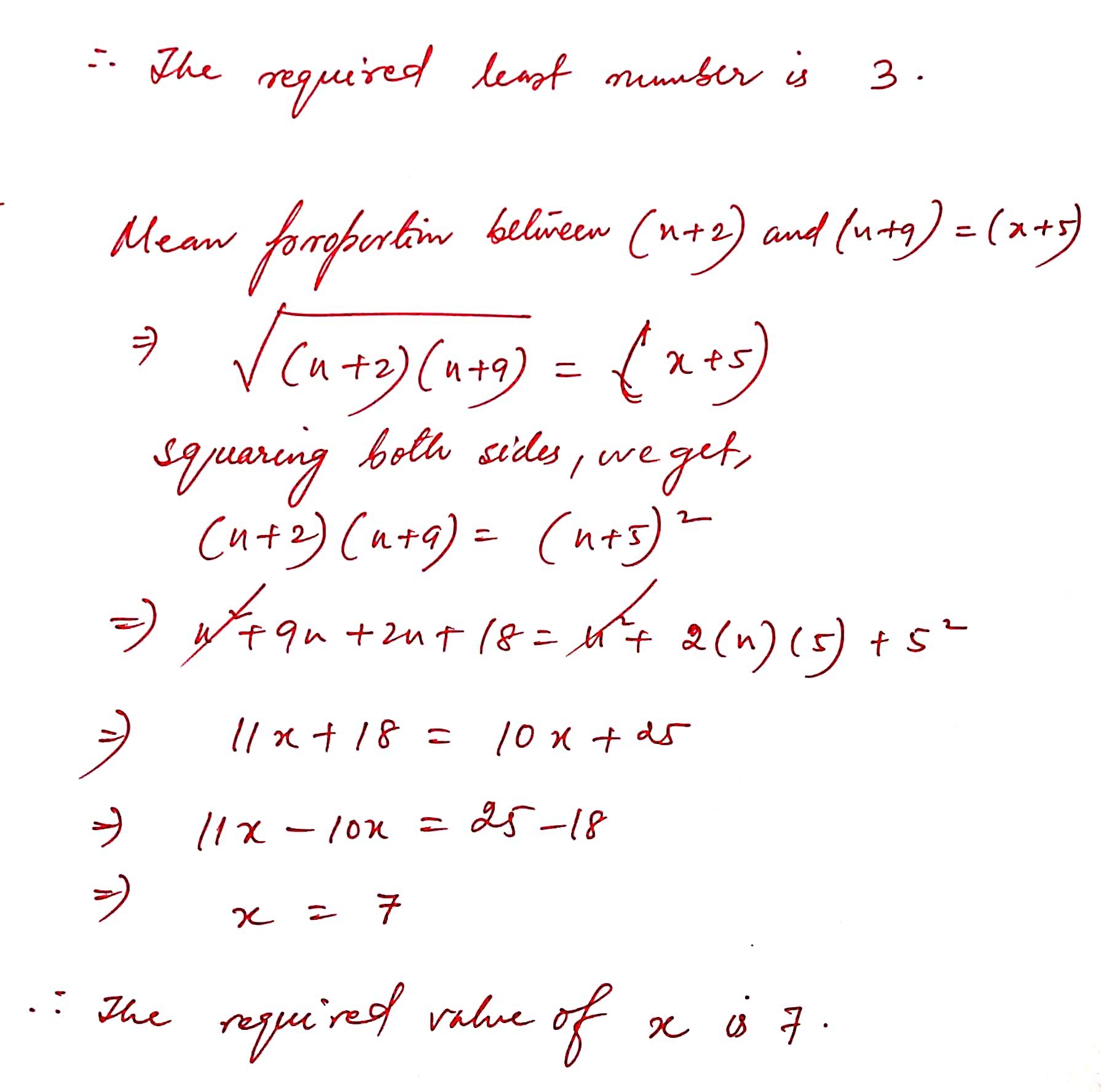1 ) Check whether the numbers 2,3,4,6 are in proportion or not.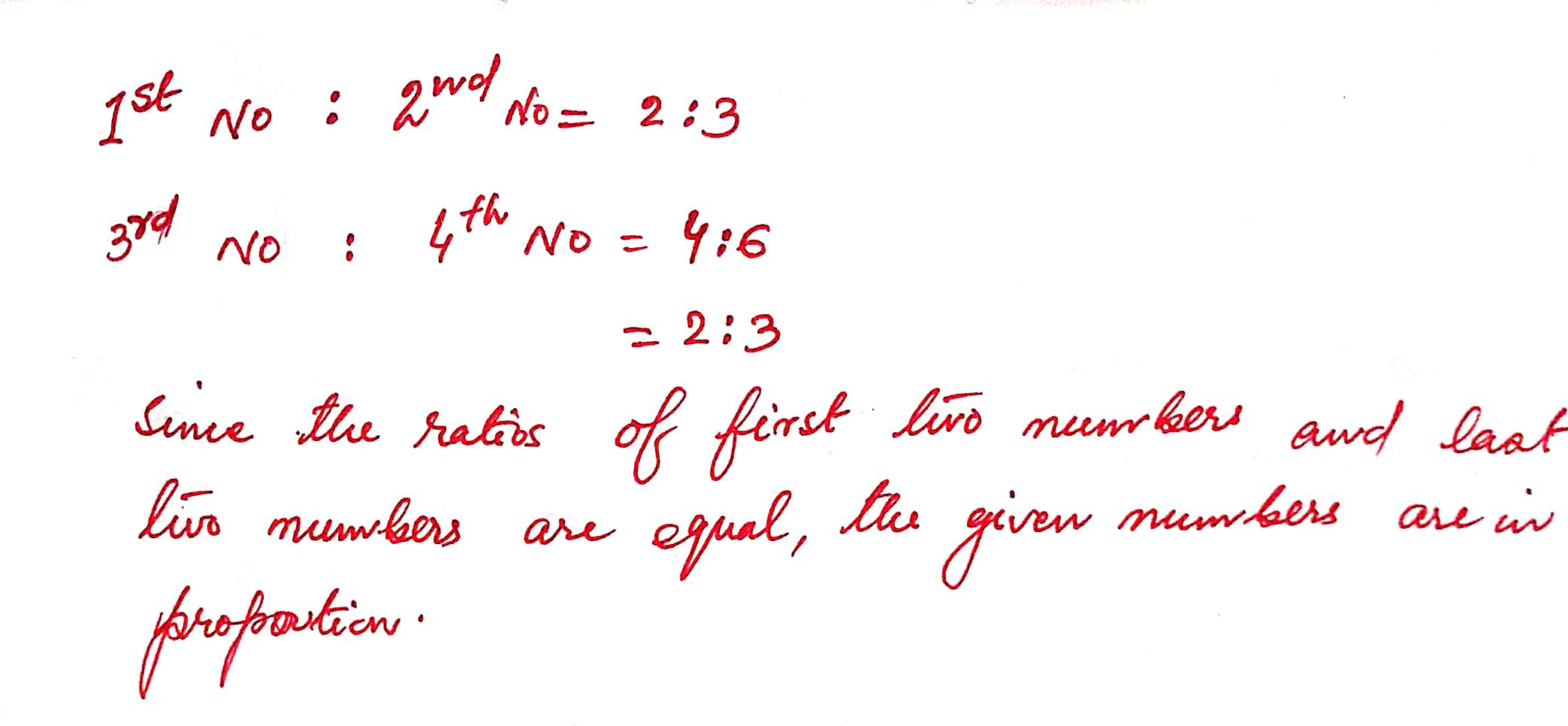2 ) Check whether the numbers 2.5,-2,-5,4 are in proportion or not.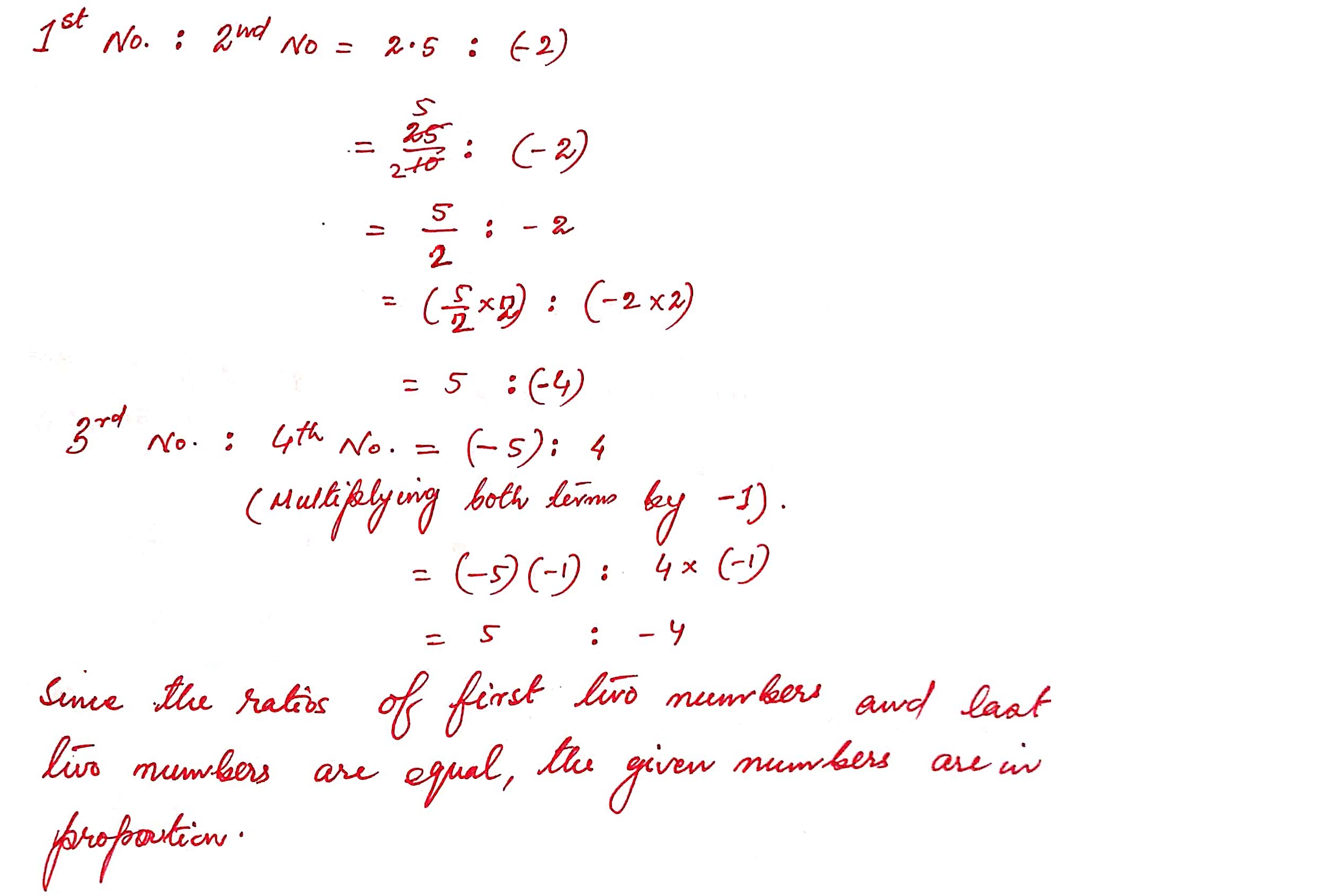3 ) Check whether the numbers 2,7,14,42 are in proportion or not.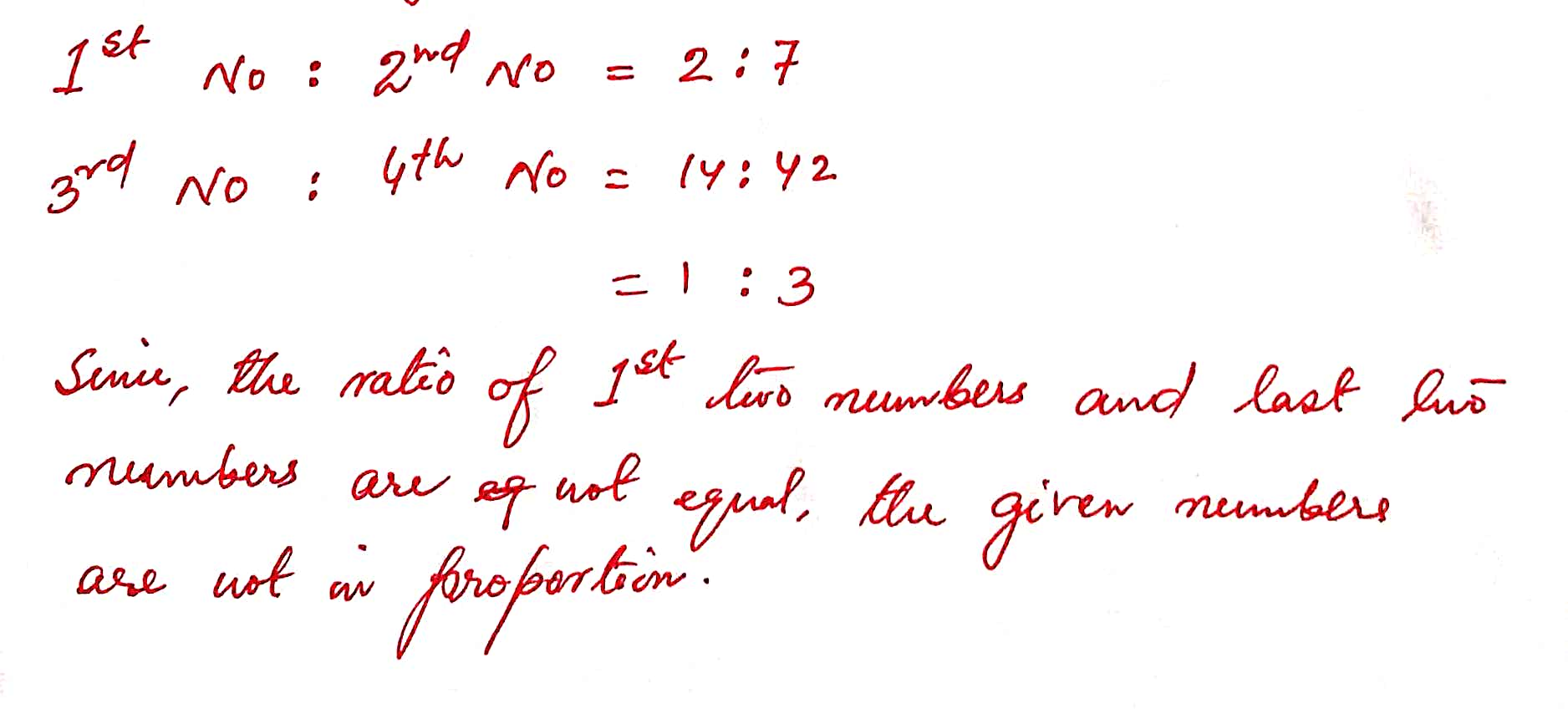4) Check whether the numbers –2,6,1,18 are in proportion or not.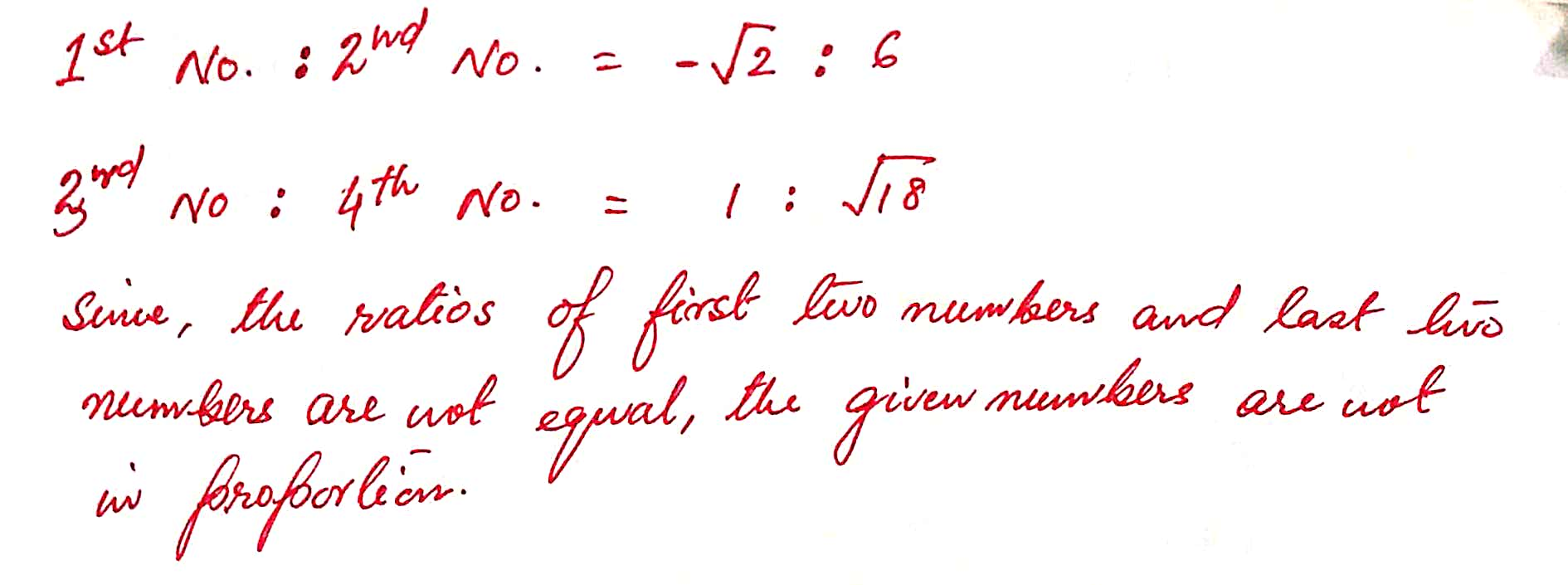5 ) Check whether the numbers 2a, 3b, 6ac, 9bc are in proportion or not.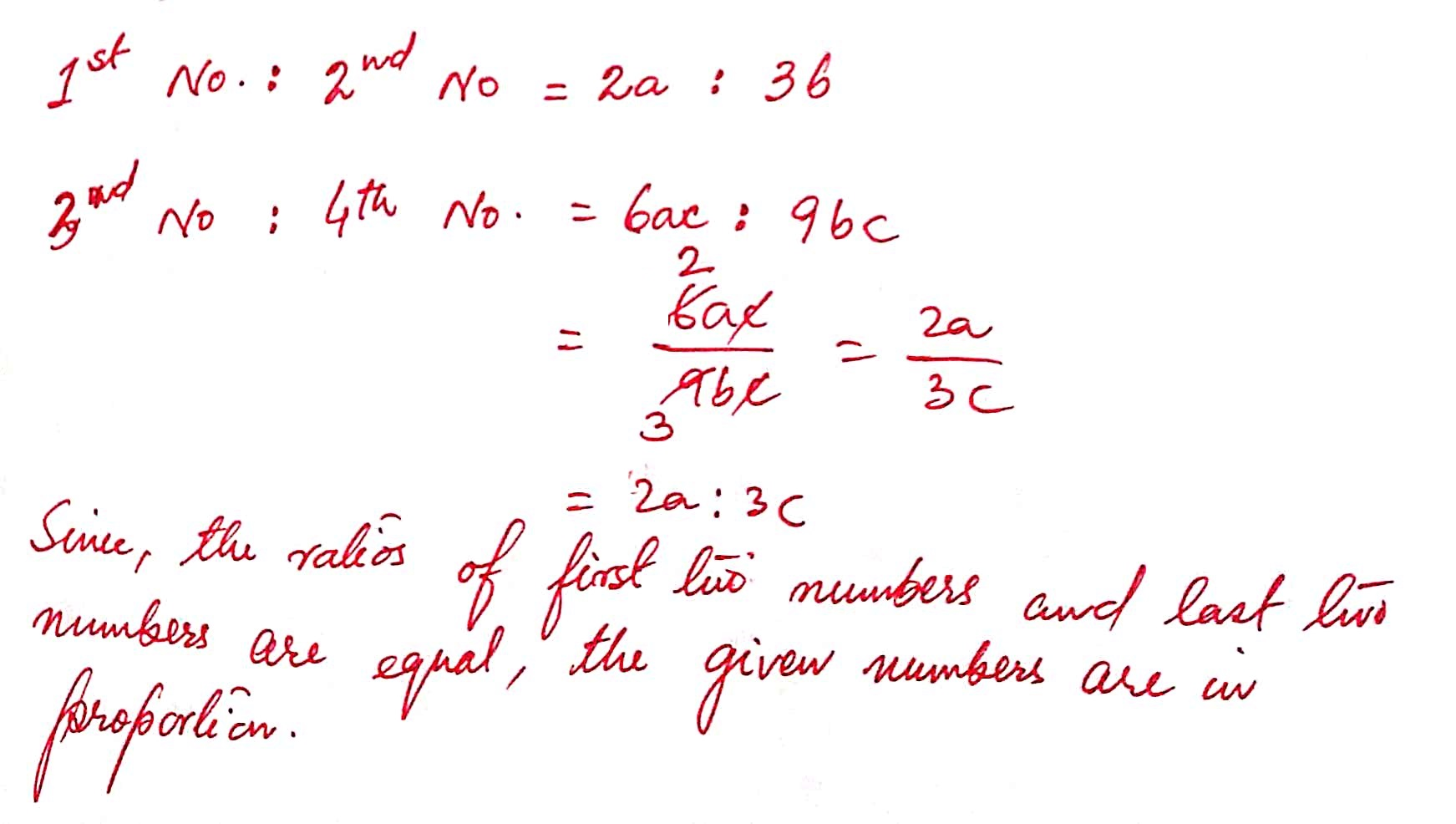6 ) Check whether the numbers 8x, 5yz, 40qx, 25qyz are in proportion or not.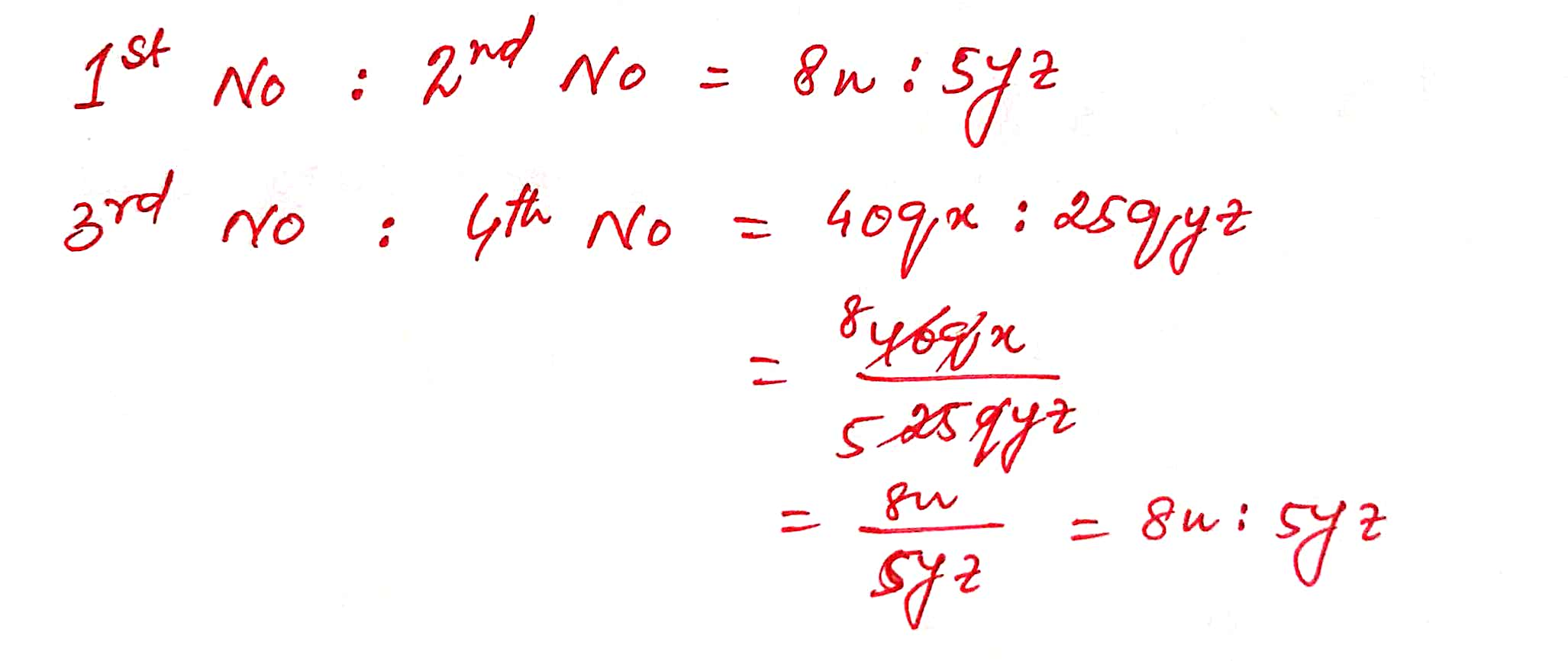7 ) Find which pair of numbers given below are proportional to 5pq and 3q ?

(i) 15pt , 3q ( ii) 15pt and 9t (iii) 15pr and 9t

8 ) If 6 : x :: 2 : 13, find the value of x.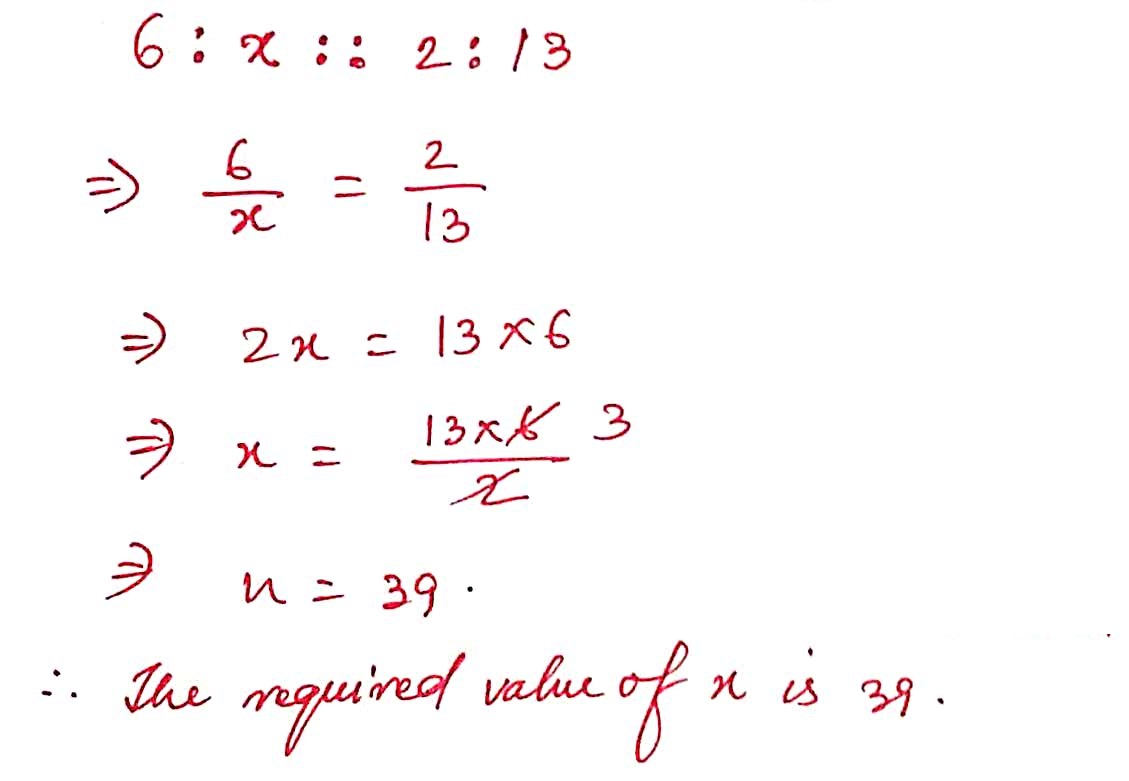9 ) If 8 : y :: 2 : 21, calculate the value of y.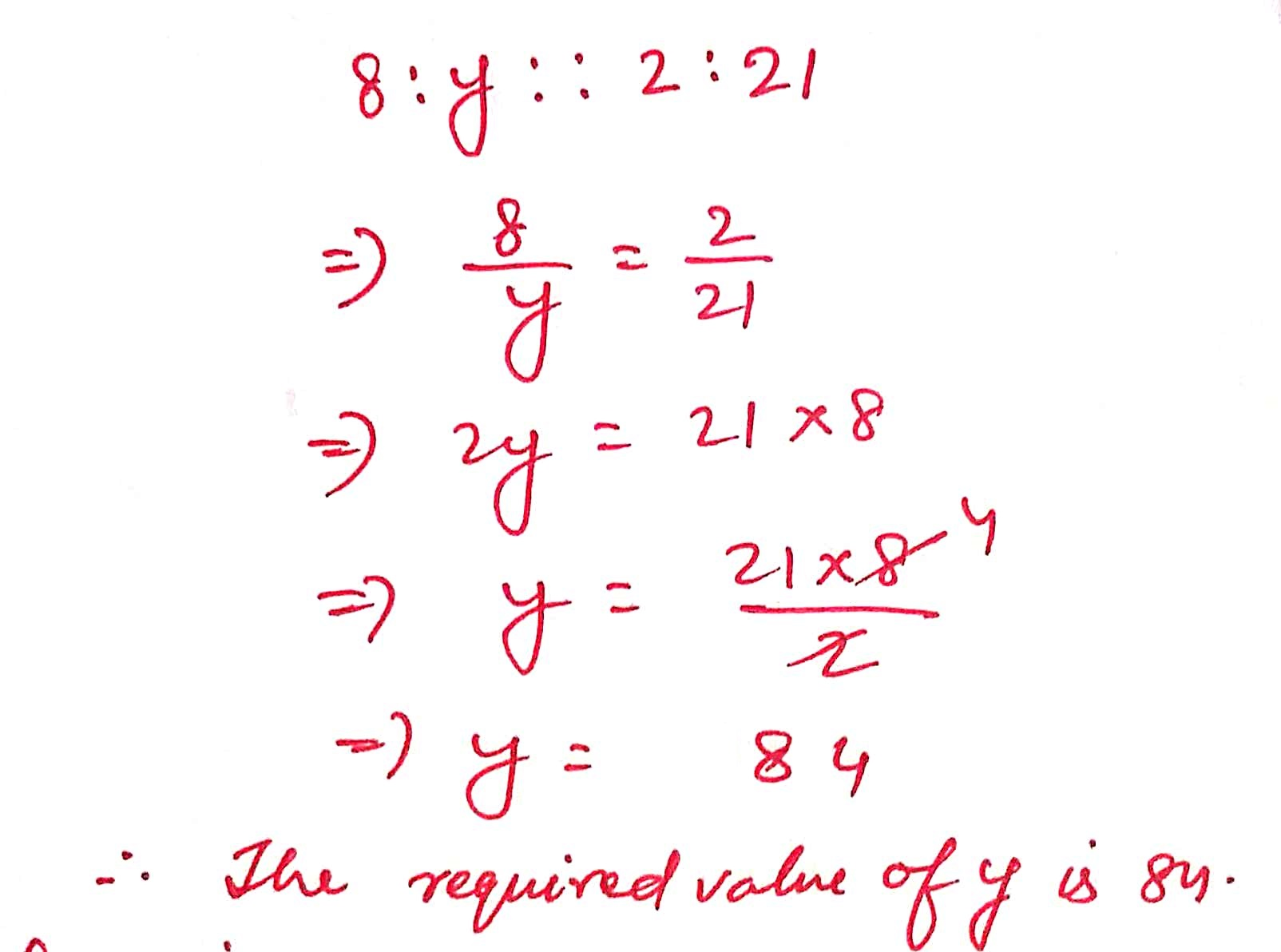10 ) Find out the value of x, if 10 : 35 :: x : 42.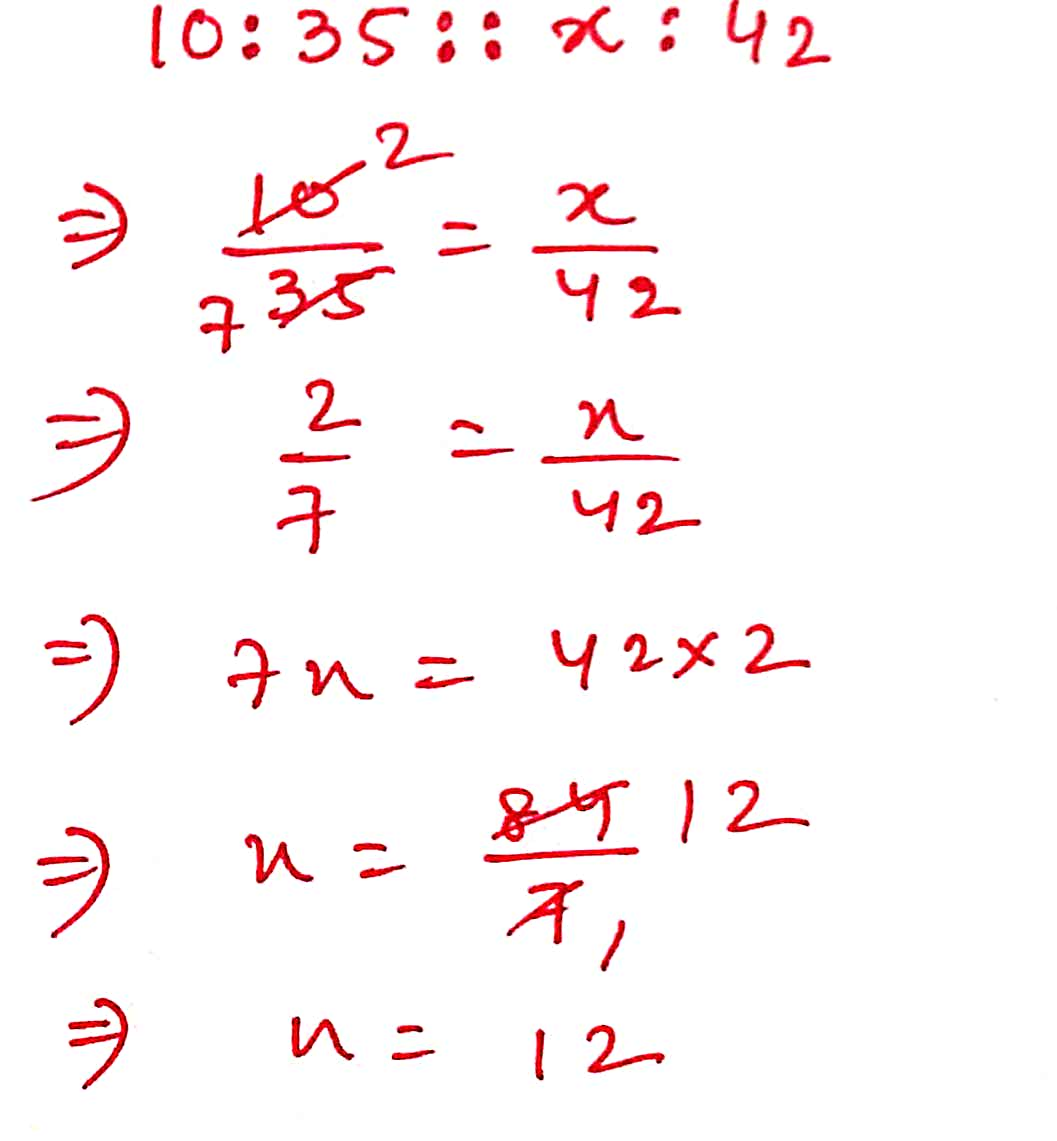11 ) For what value of x, x, 50 , 3 and 2 will be in proportion ?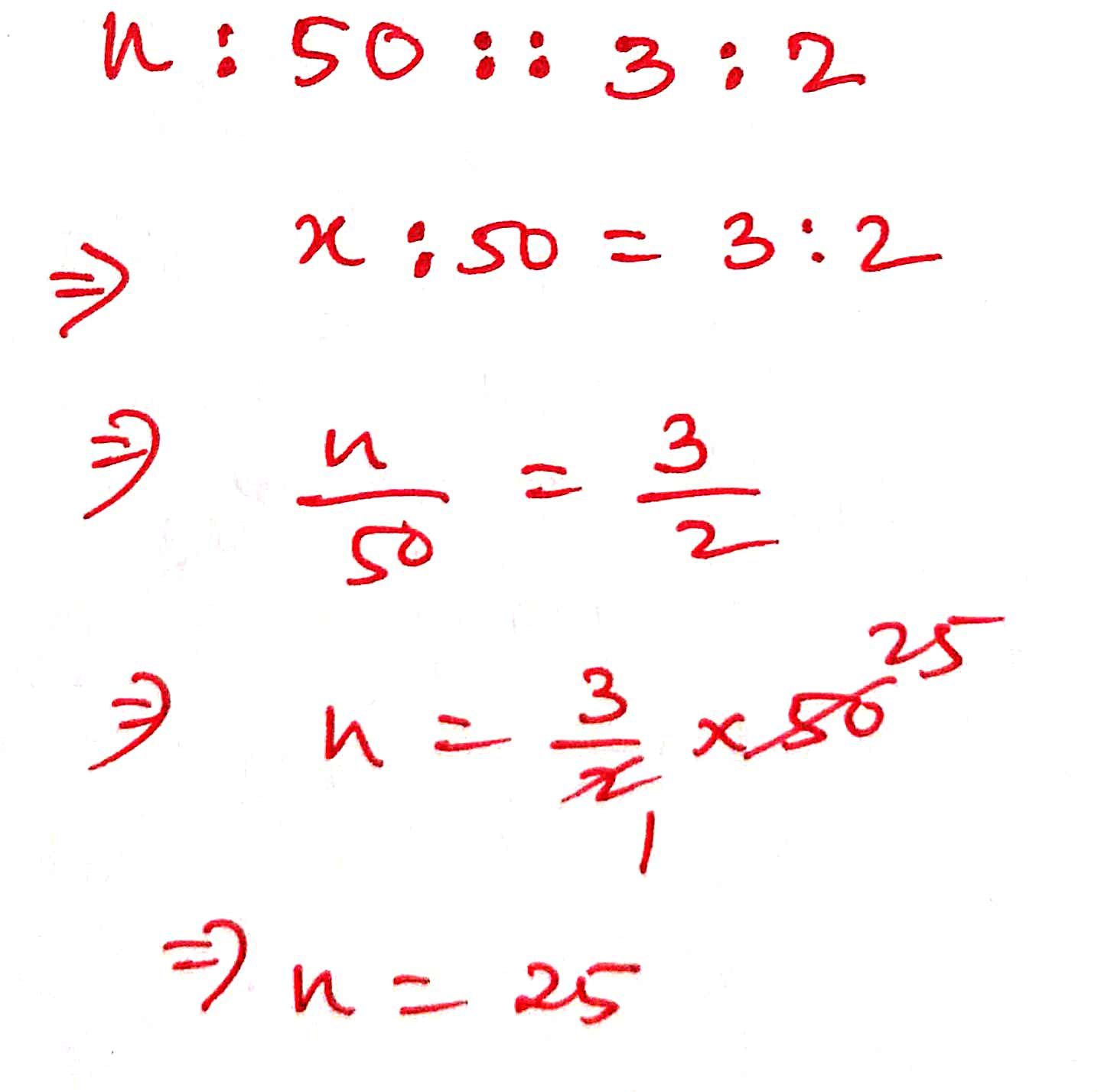12 ) Find the fourth proportional of 1.5, 4.5, and 3.5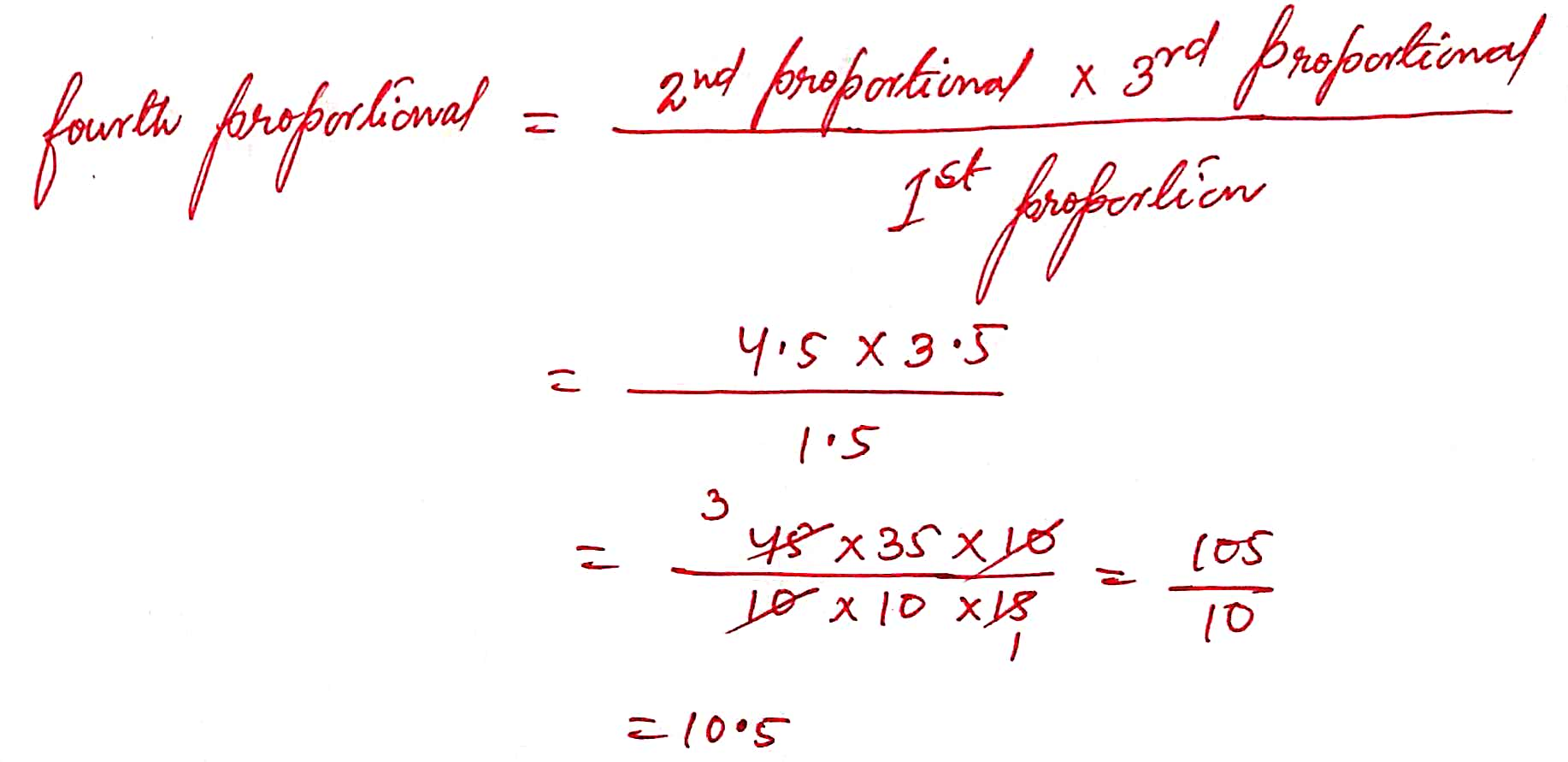13 )Find the fourth proportional of 3a, 6a2 , 2ab2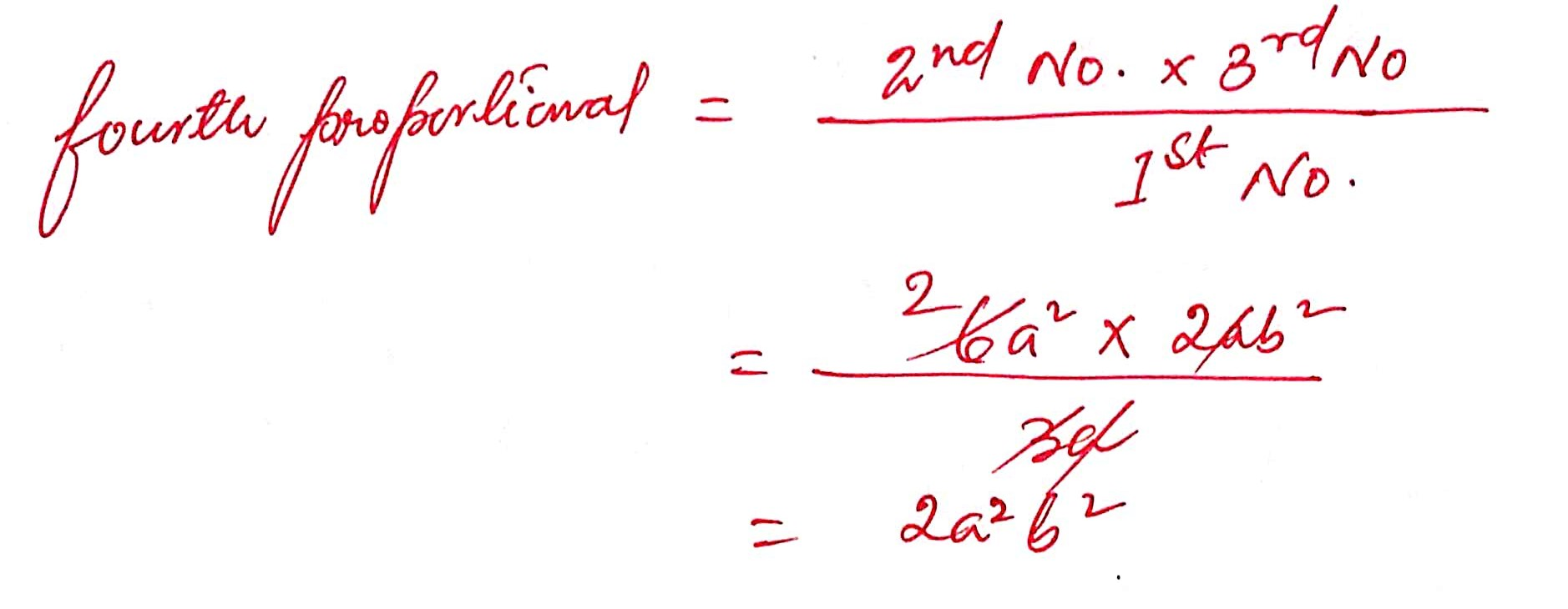14 ) Find the fourth proportional of 1/3, 1/4, 1/5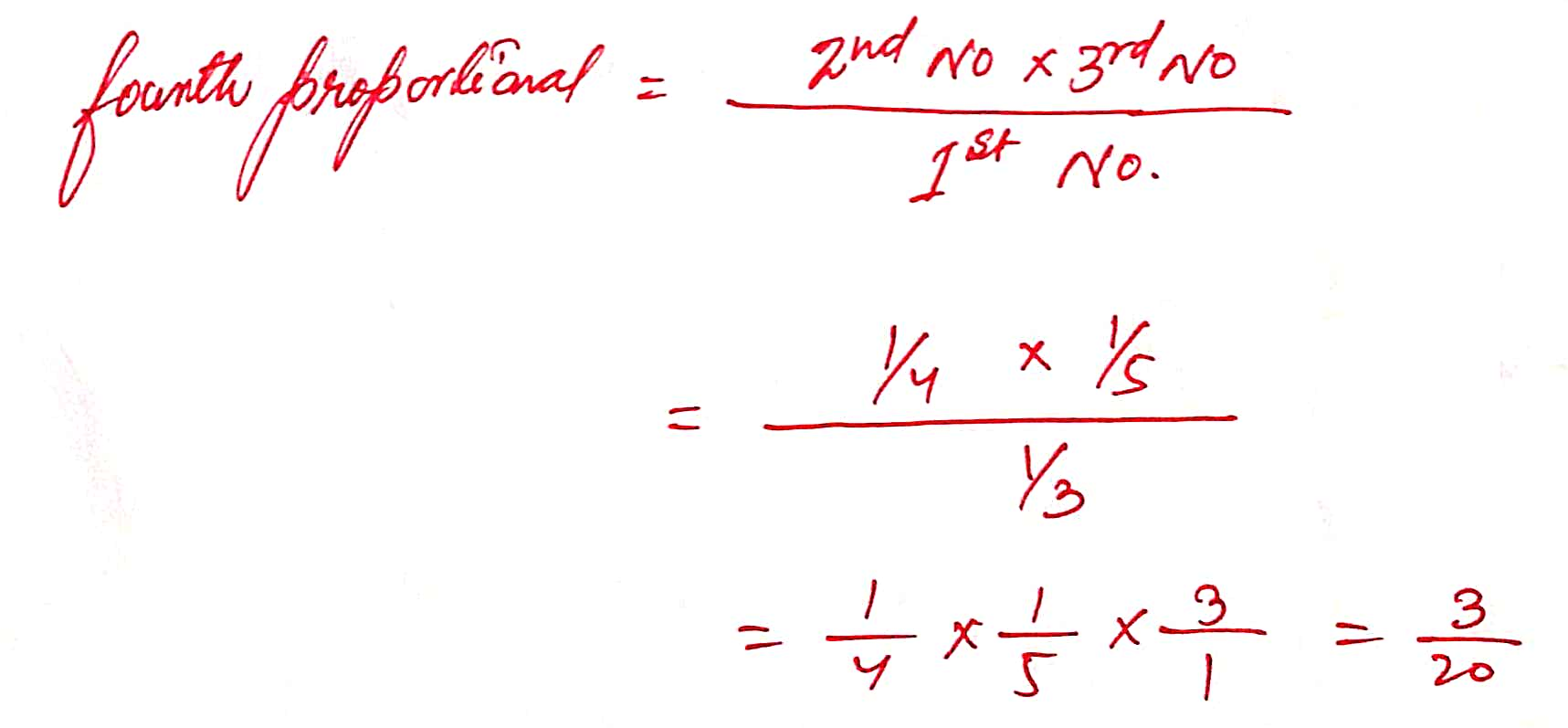15 ) Find the fourth proportional of 9.6 Kg, 7.6 kg and 28.8 kg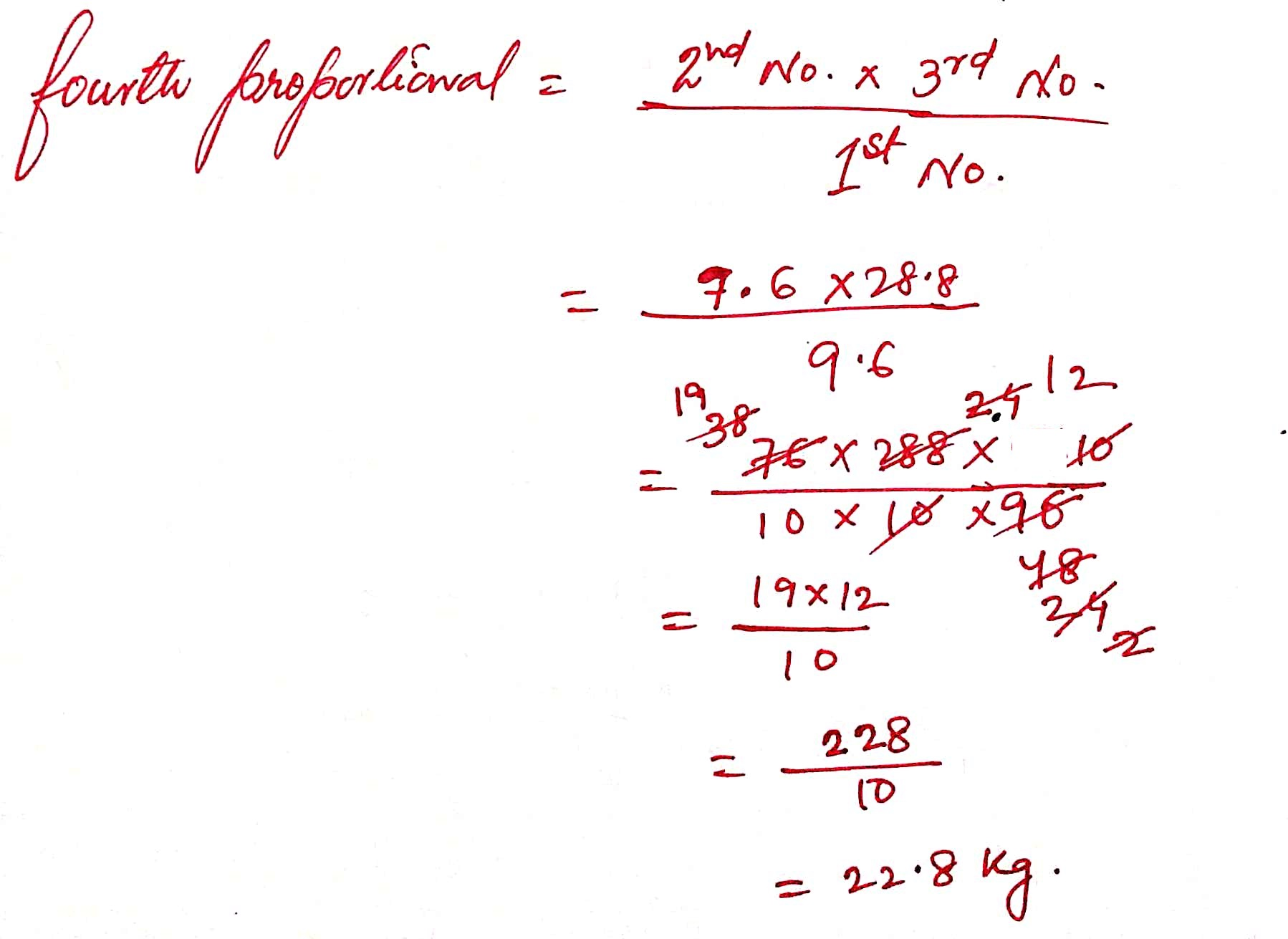16 )Find the fourth proportional of ( p – q ), ( p2 – q2 ), ( p2 – pq + q2 )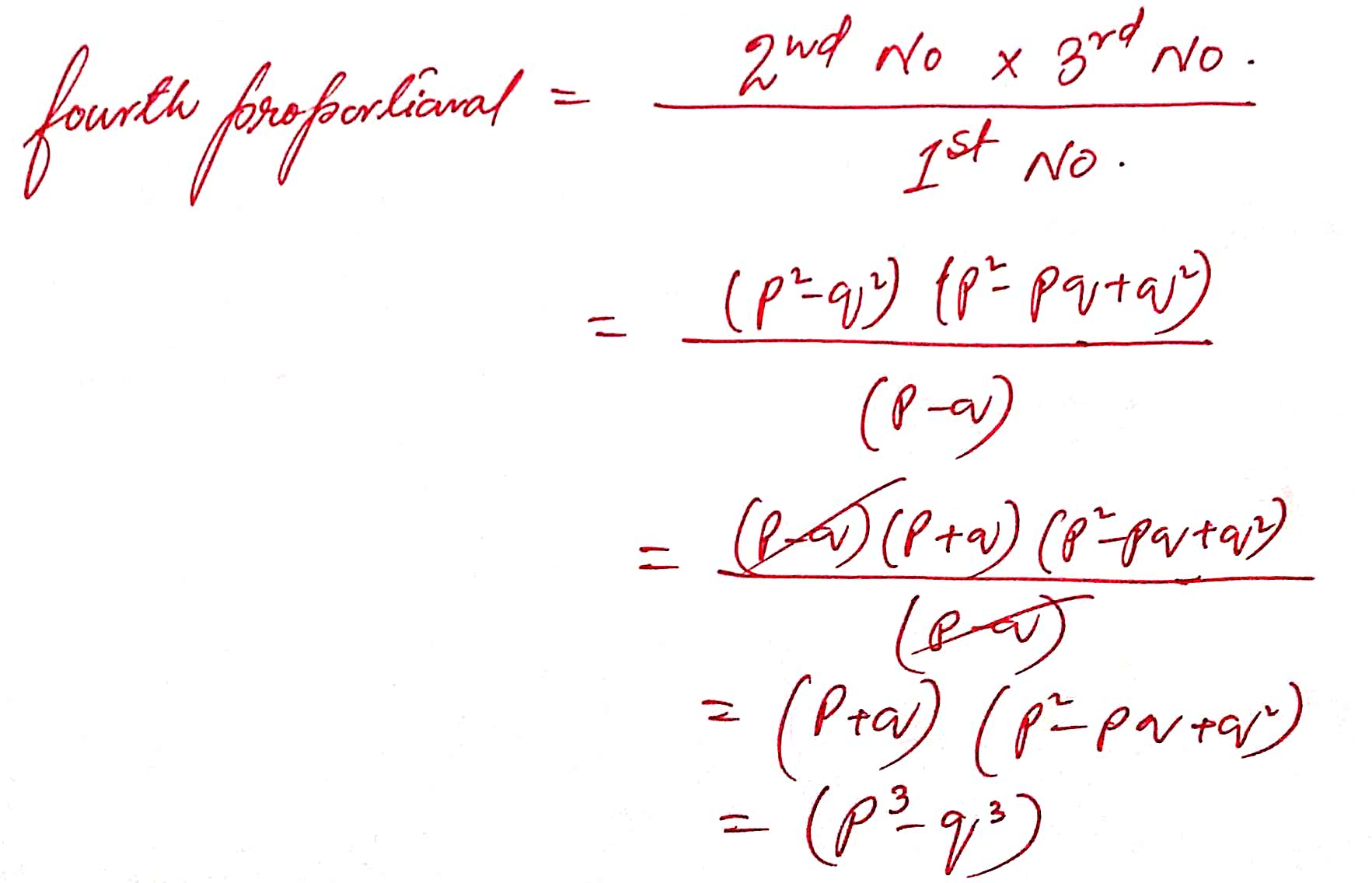17) Find the fourth proportional of x2y, y2z , z2x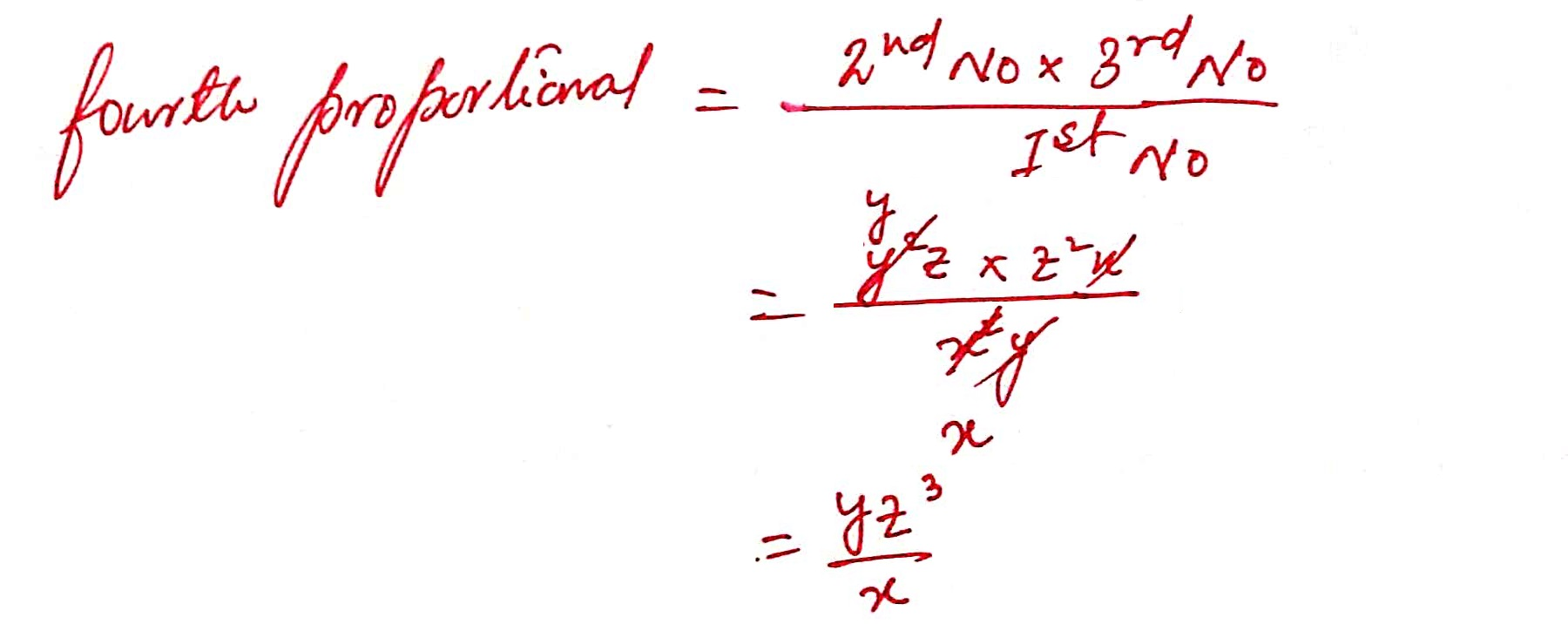18 ) Find the fourth proportional of 5, 4, 25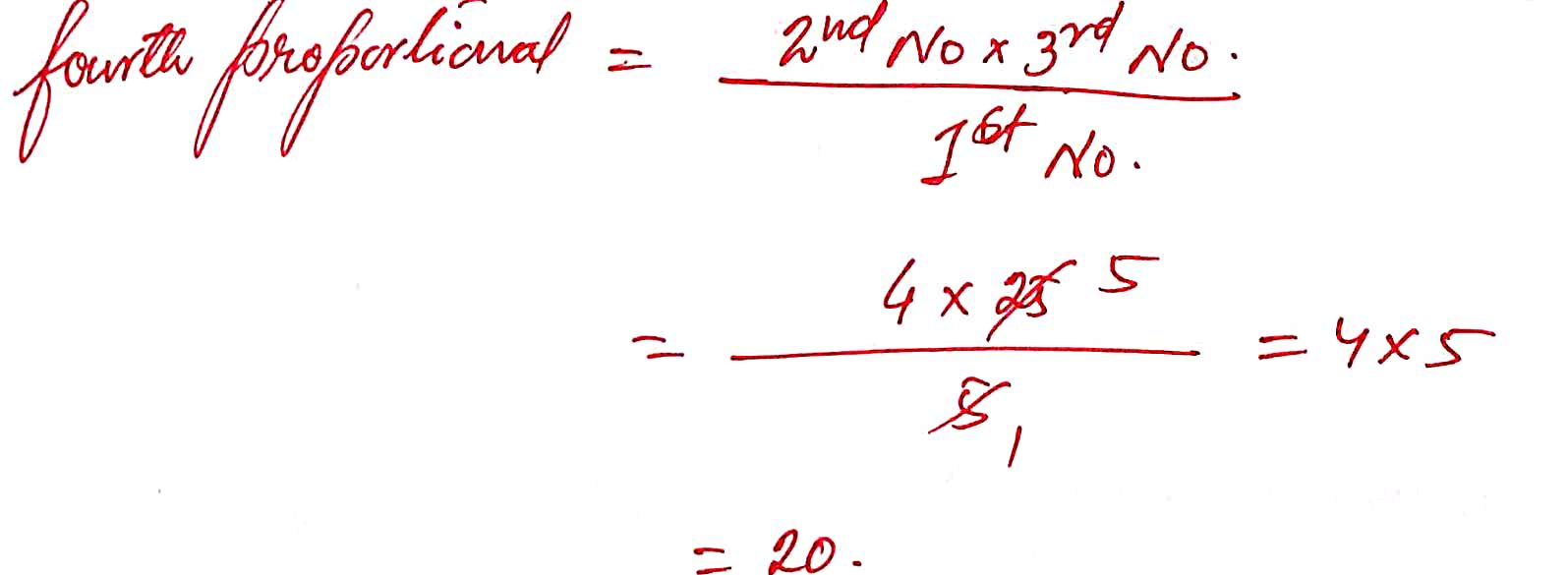19 ) Find the third proportion of the numbers 9 and 15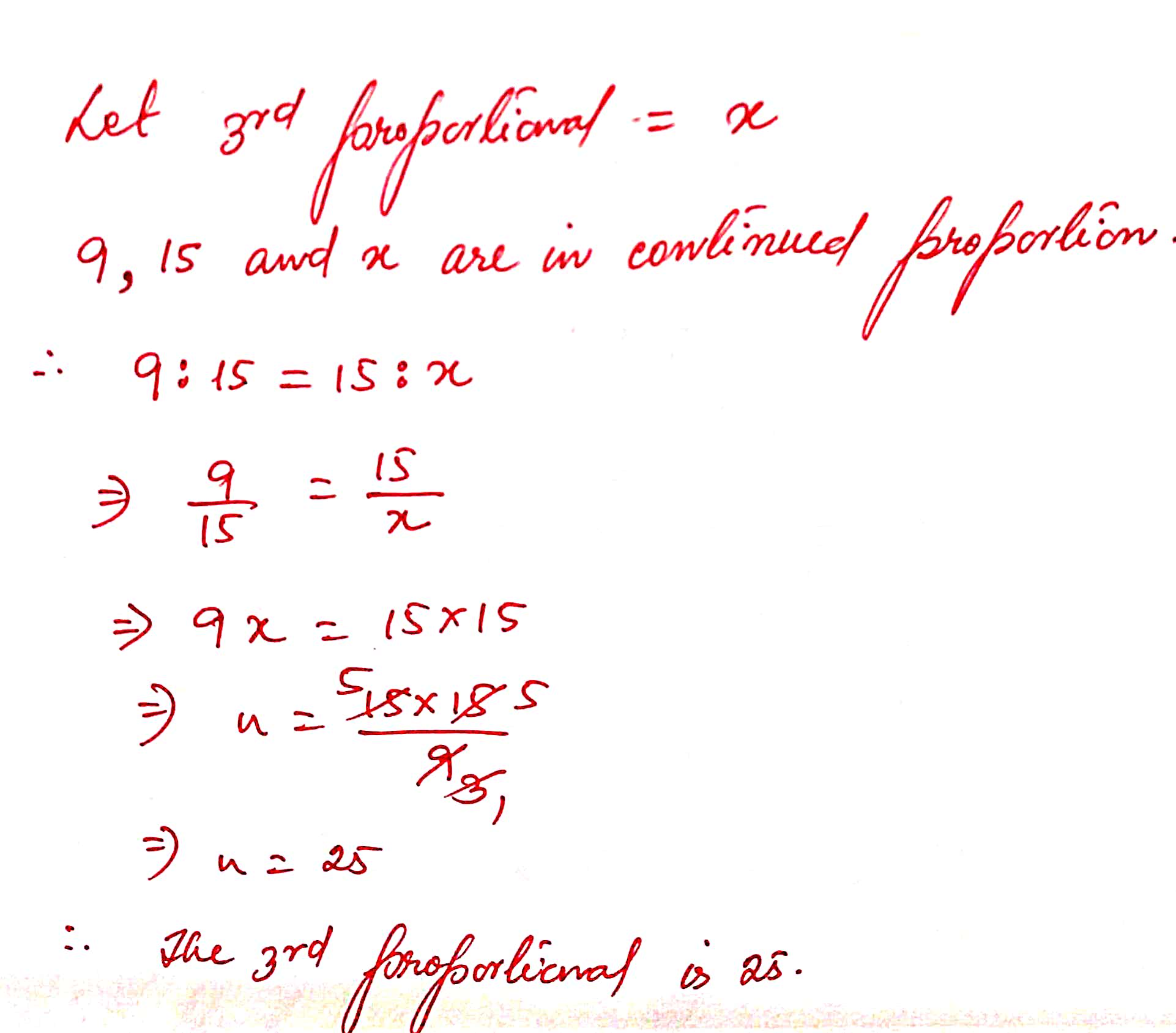20 ) Find the third proportion of the numbers 2a2 and 3ab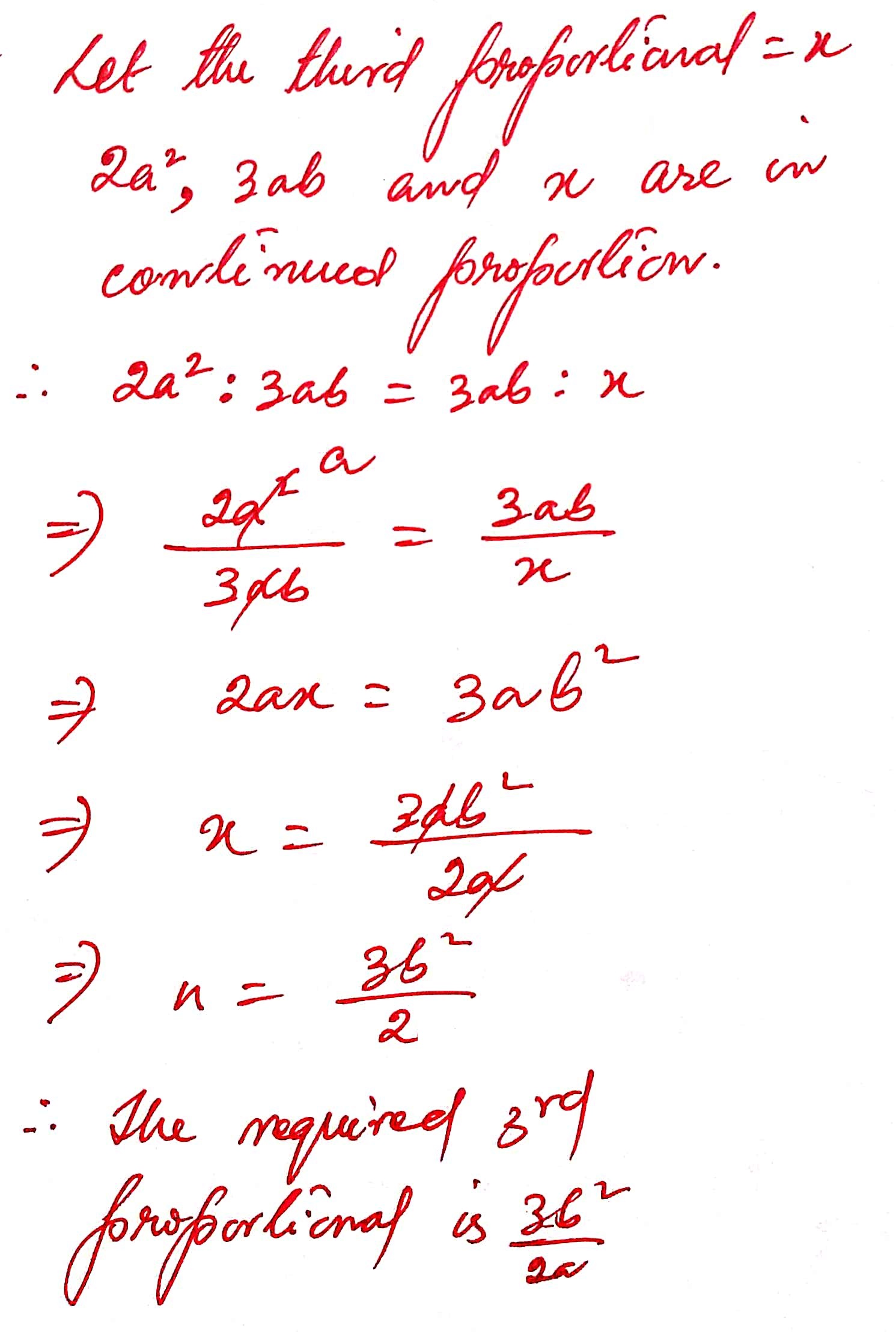21 ) Find the third proportion of the numbers 9pq and 12pq2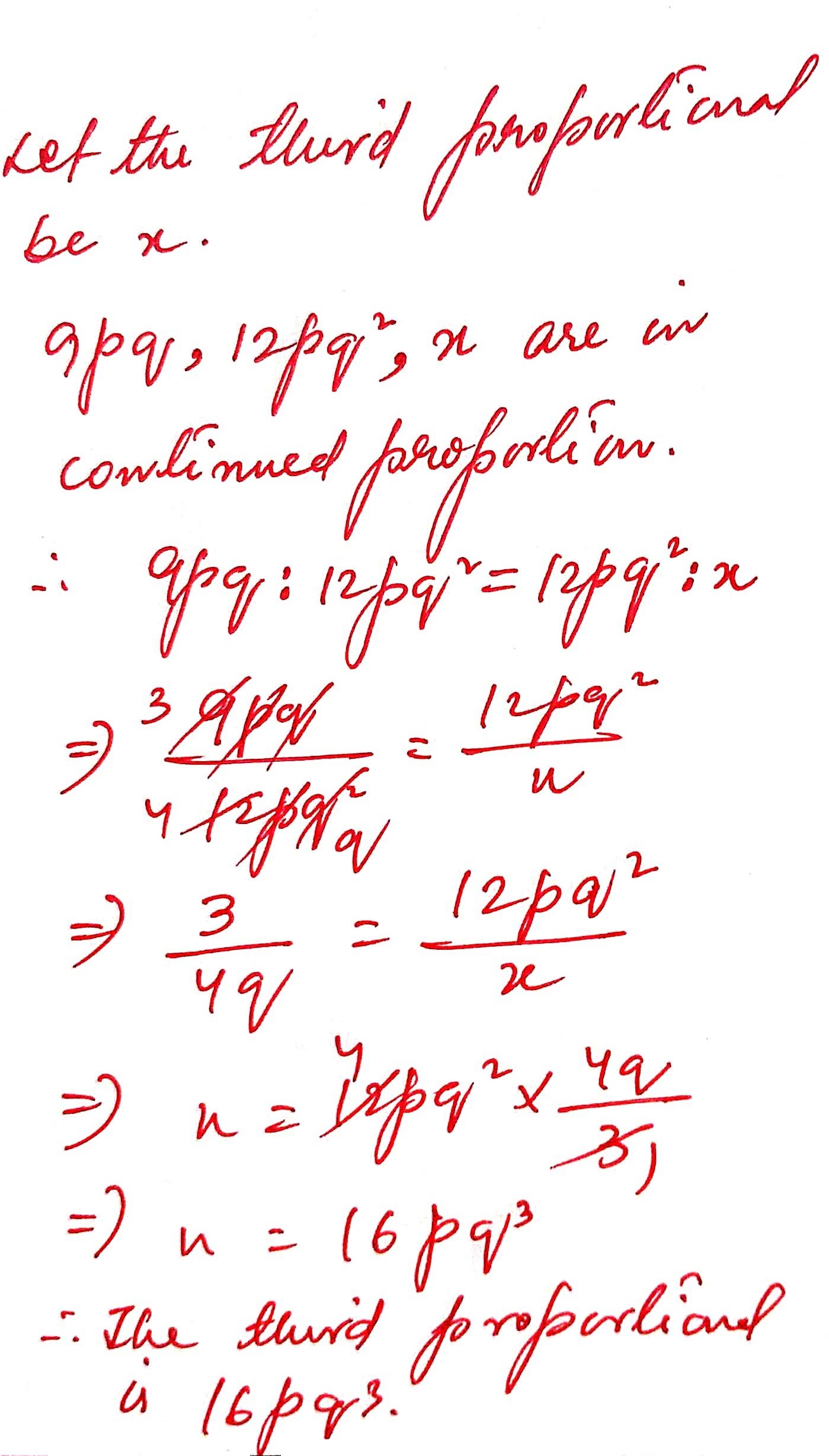22 ) Find the third proportion of the numbers 1/12 and 1/75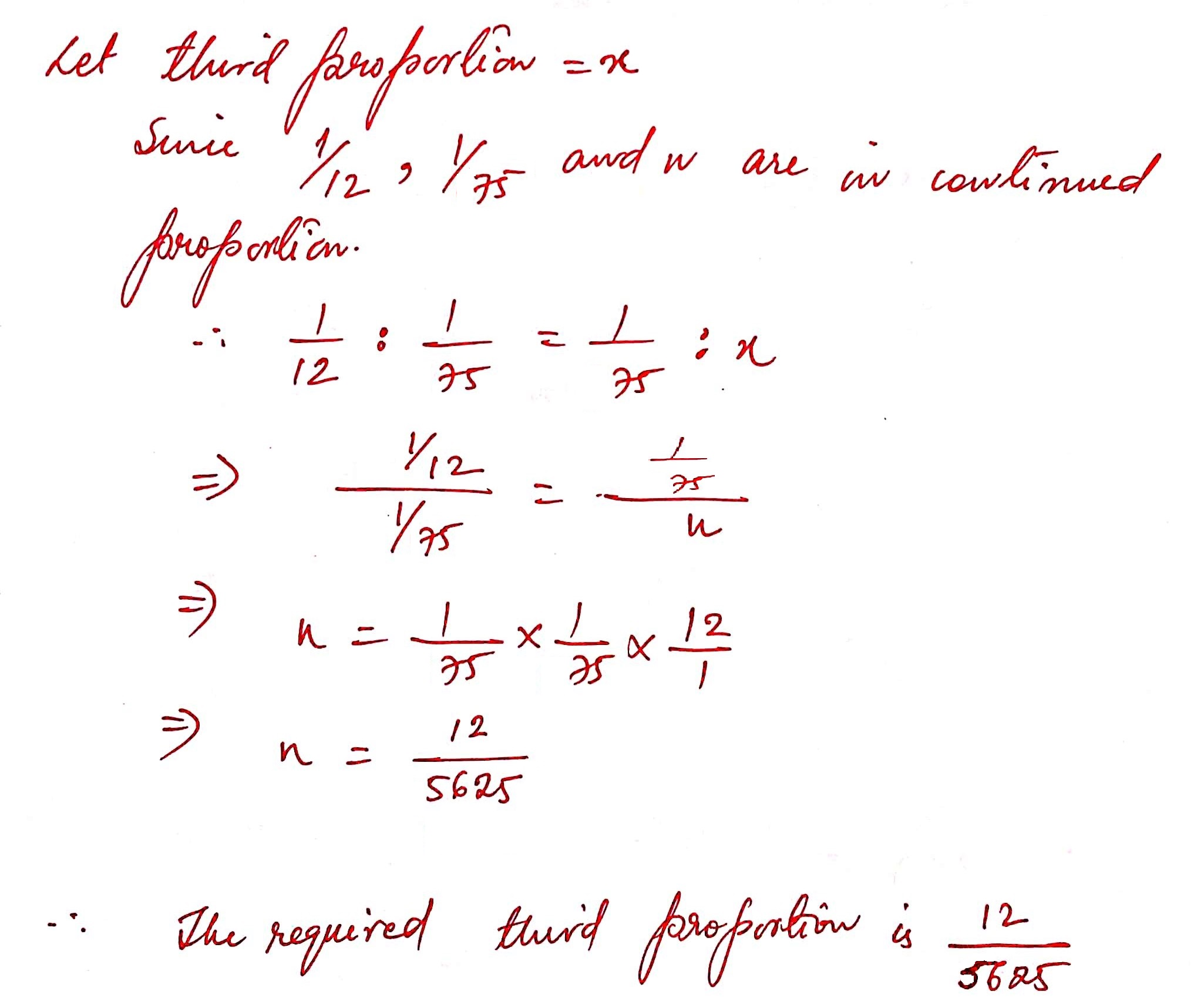23 ) Find the third proportion of the numbers 2 2/3 and 4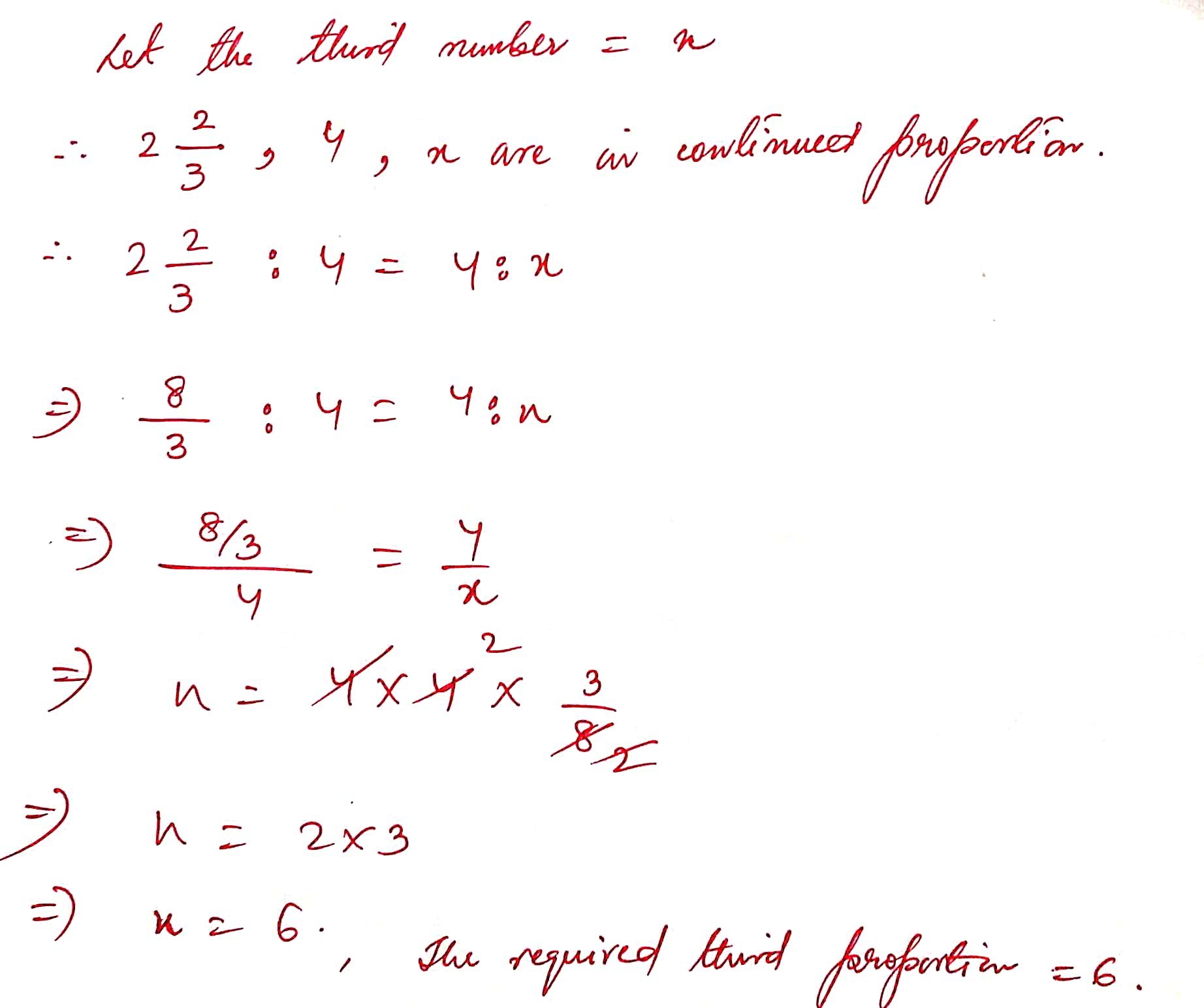24 ) Find the third proportion of the numbers ( a – b ) and ( a2 – b2 )25 ) Find the third proportion of the numbers 5 and 10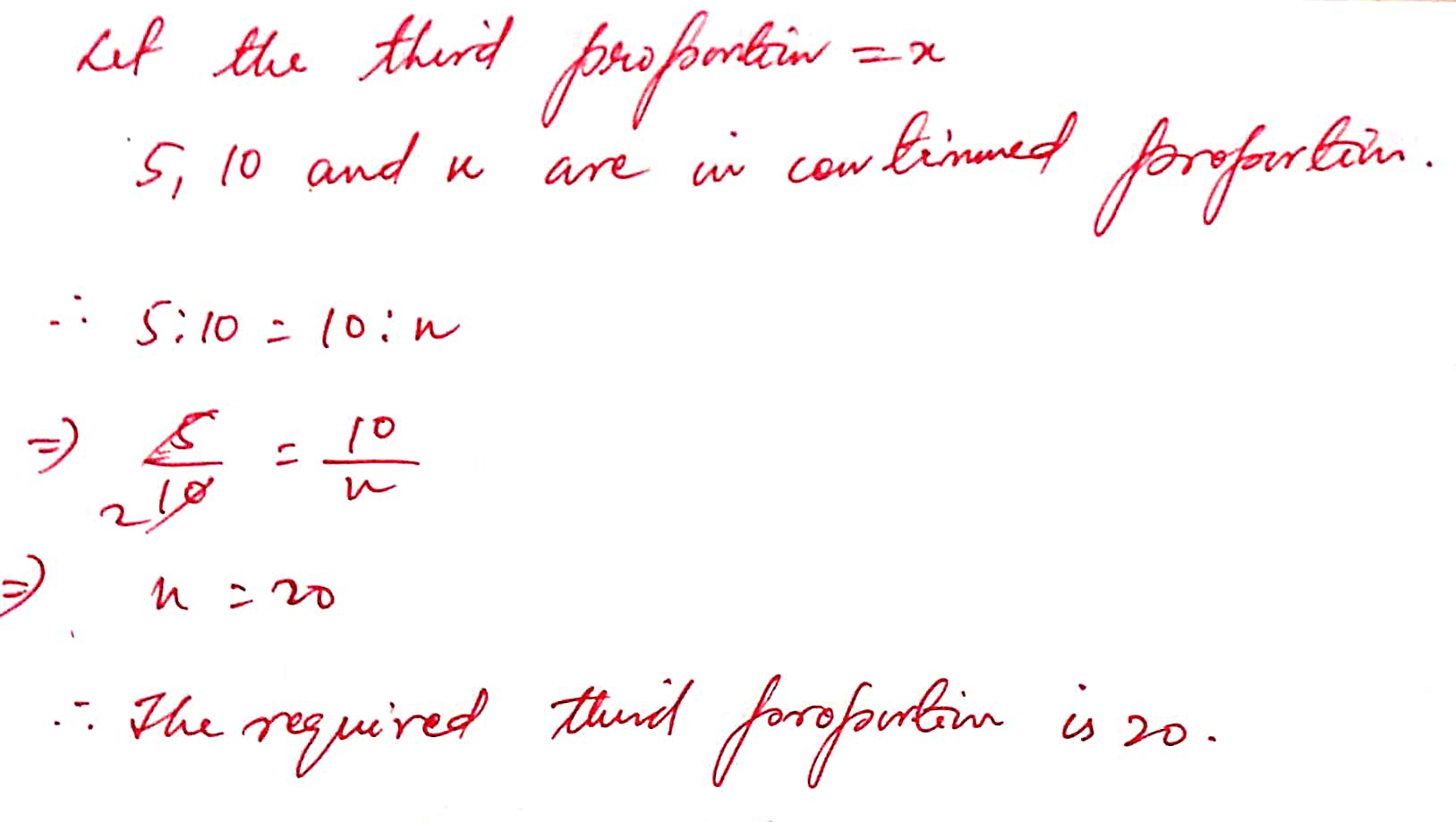26 ) Find the third proportion of the numbers 0.24 and 0.6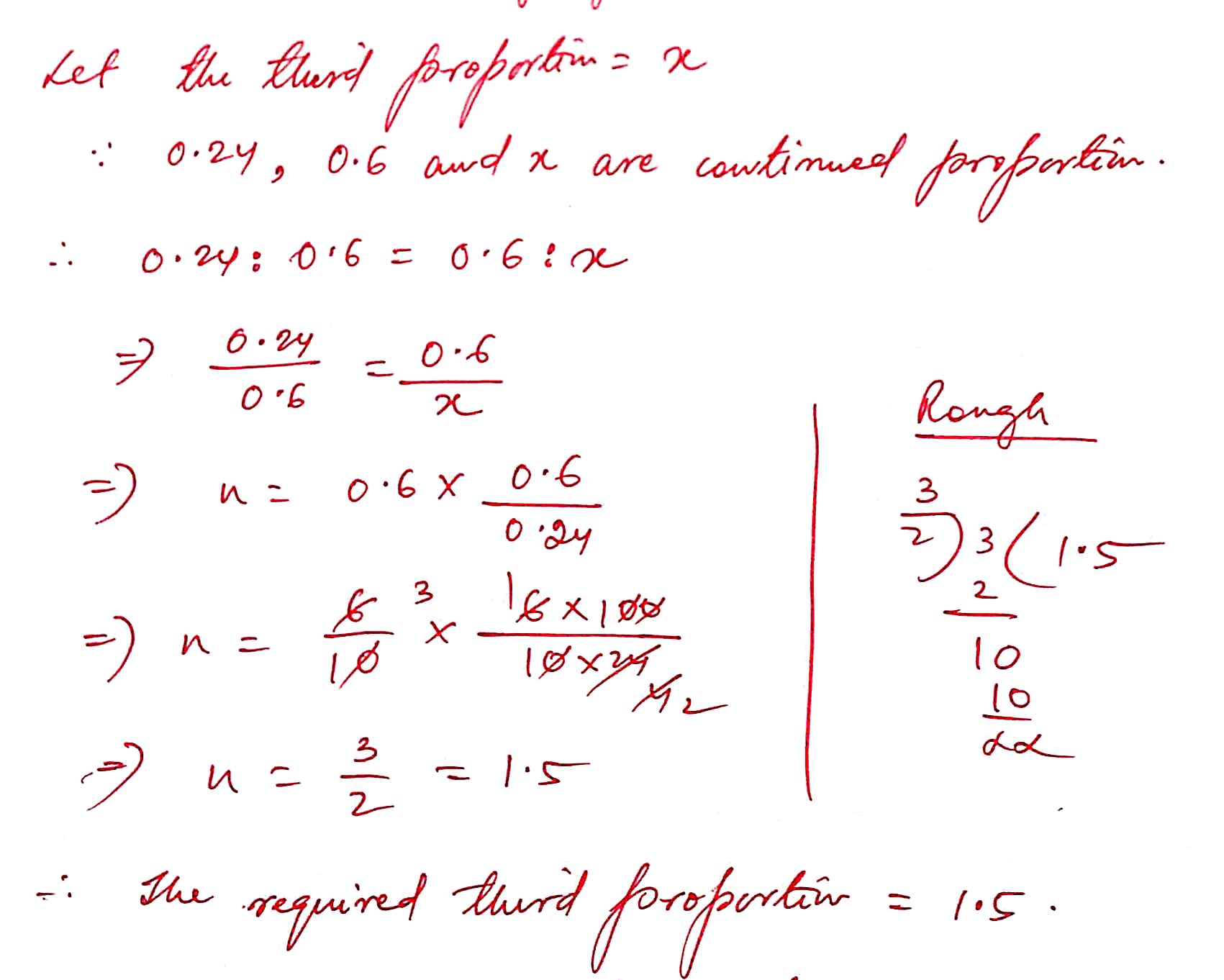27 ) Find the third proportion of the numbers p3q2 and q2r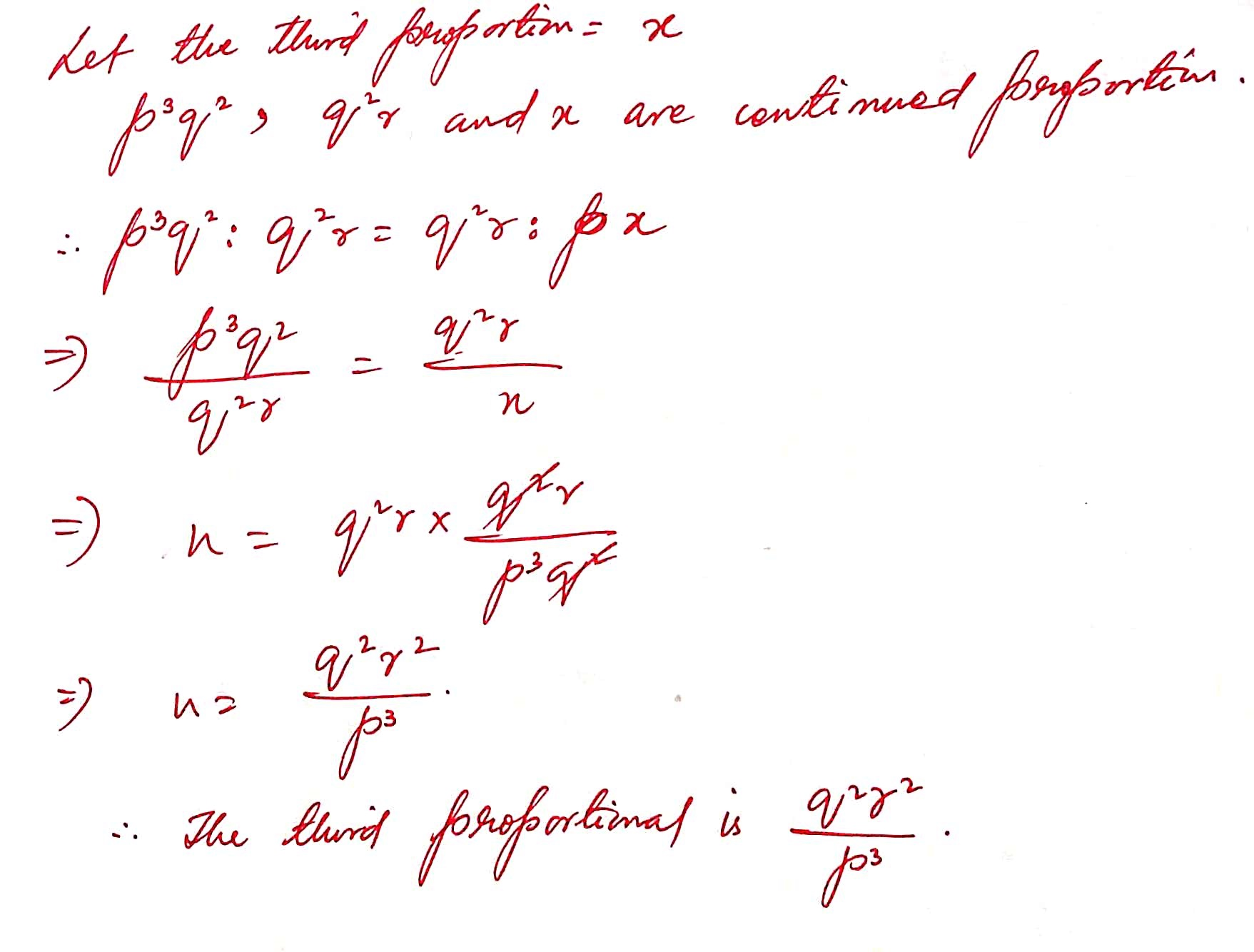28 ) Find the third proportion of the numbers ( x – y )2 and ( x2 – y2 )2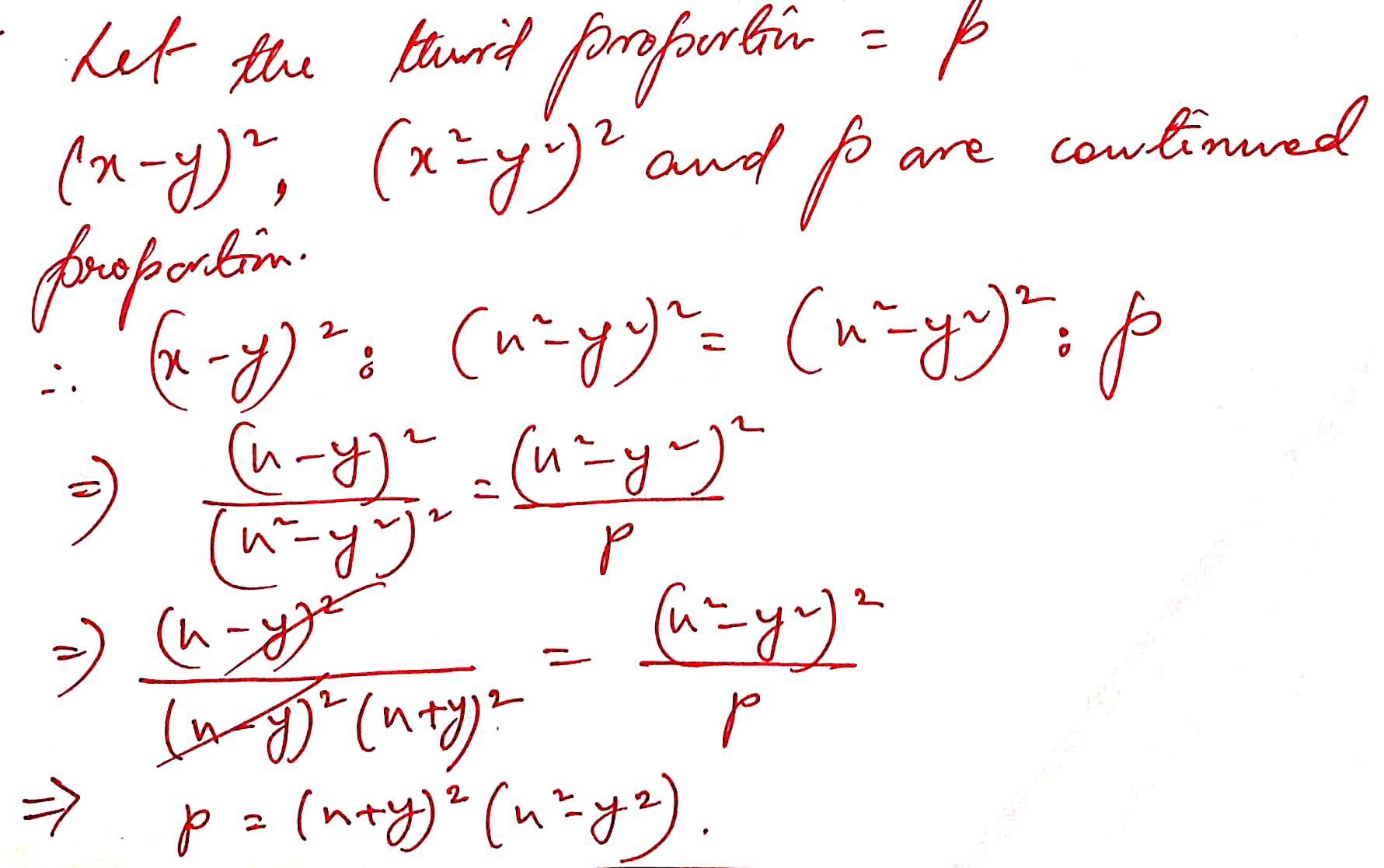29 ) Find the mean proportion of the numbers 5 and 80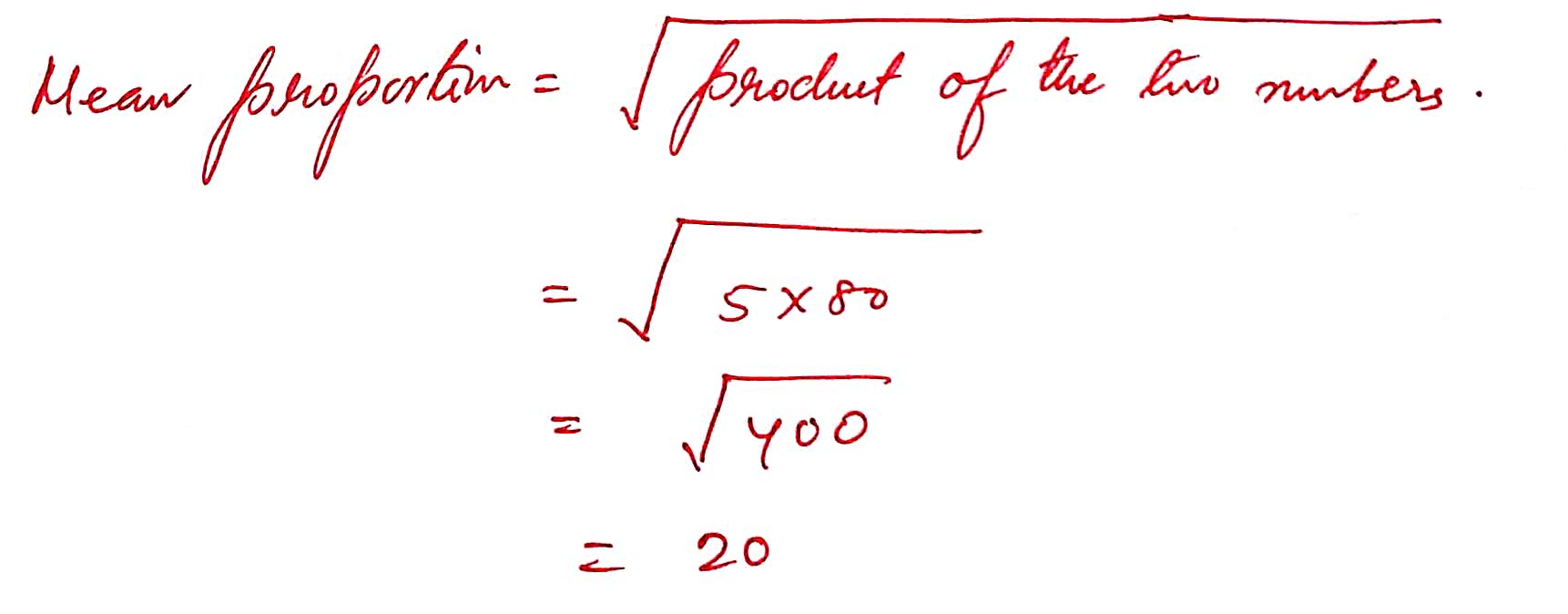30 ) Find the mean proportion of the numbers 8.1 and 2.5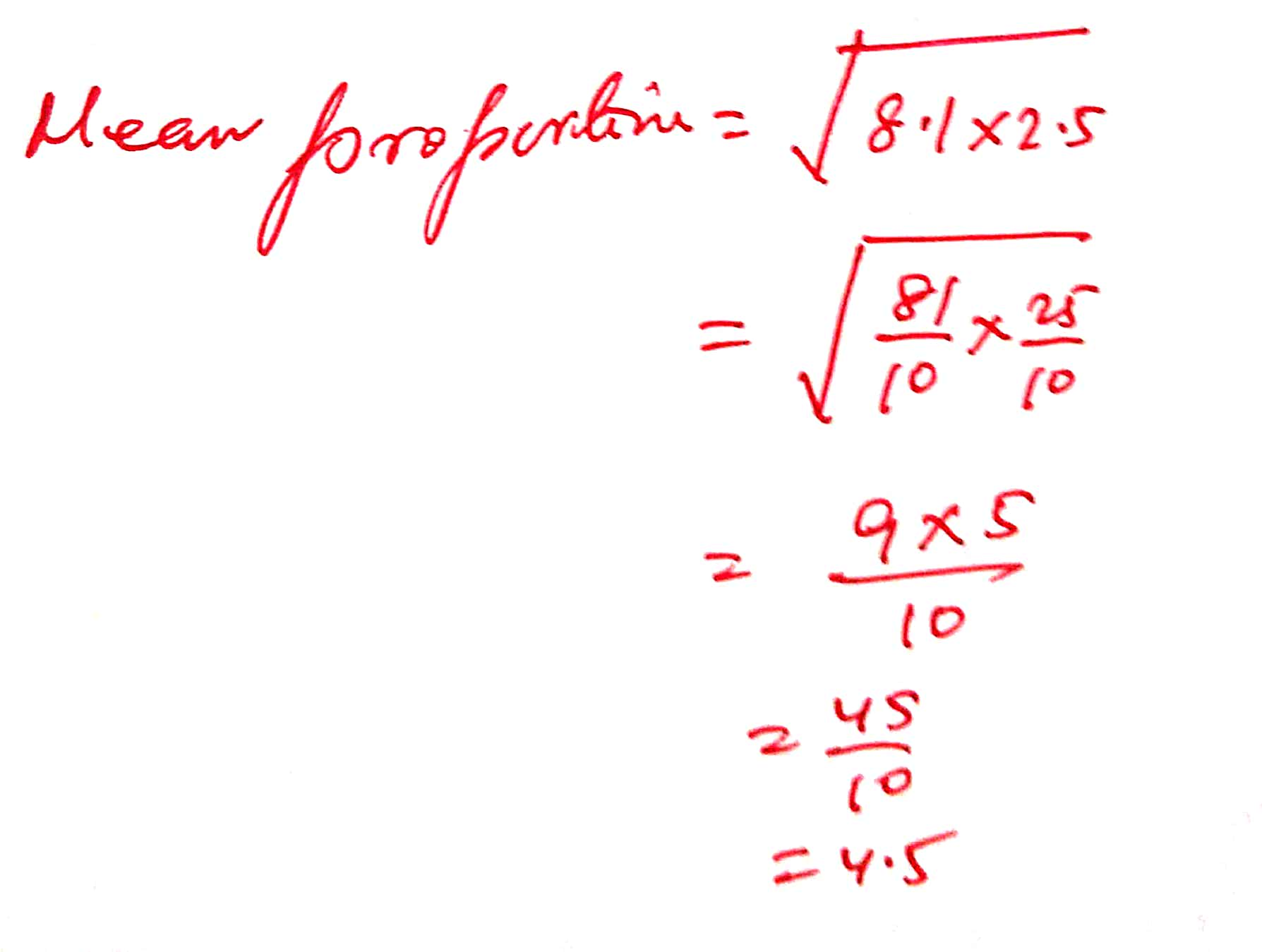31 )Find the mean proportion of the numbers x3y and xy3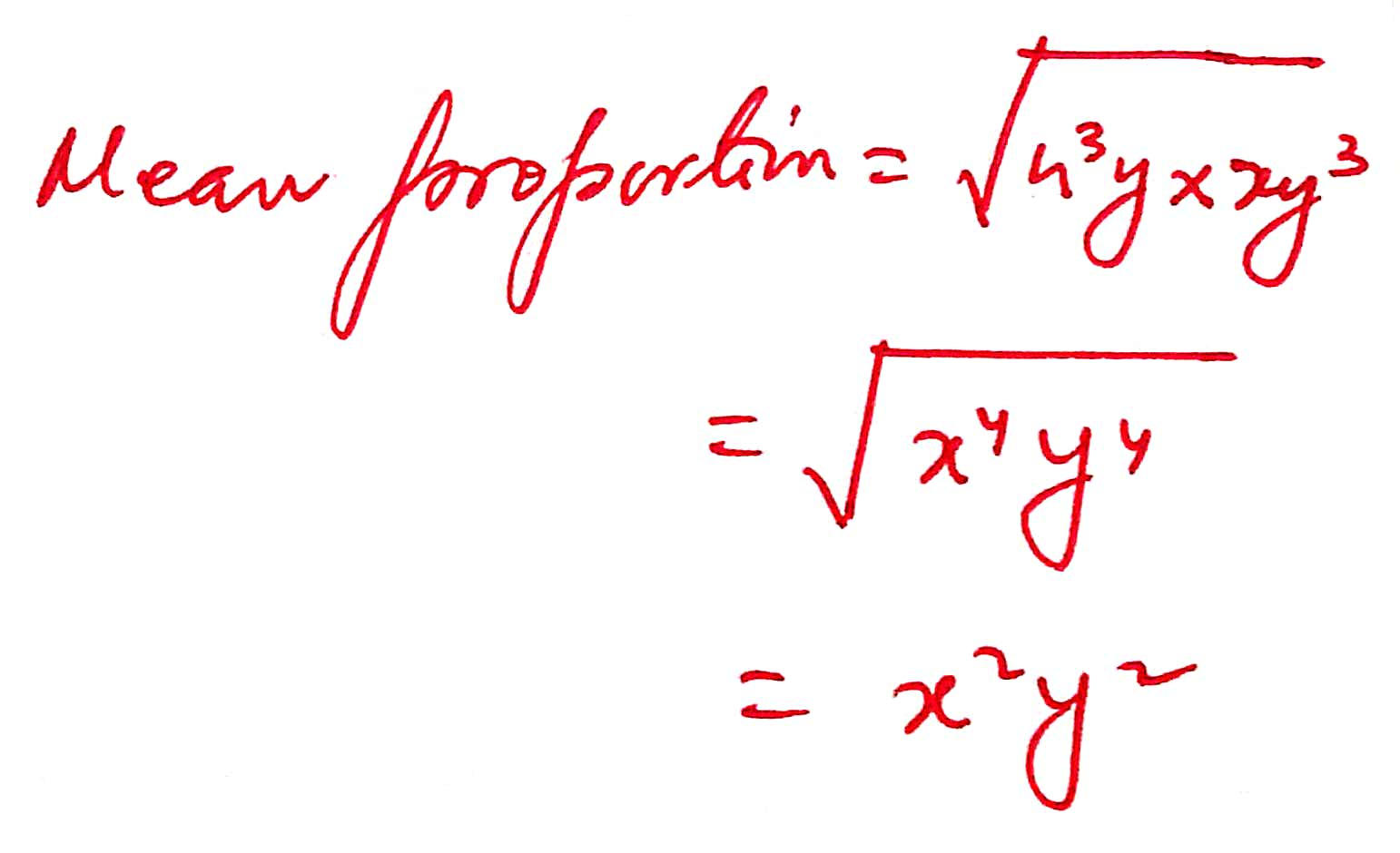32)Find the mean proportion of the numbers (x-y)2 and ( x + y )2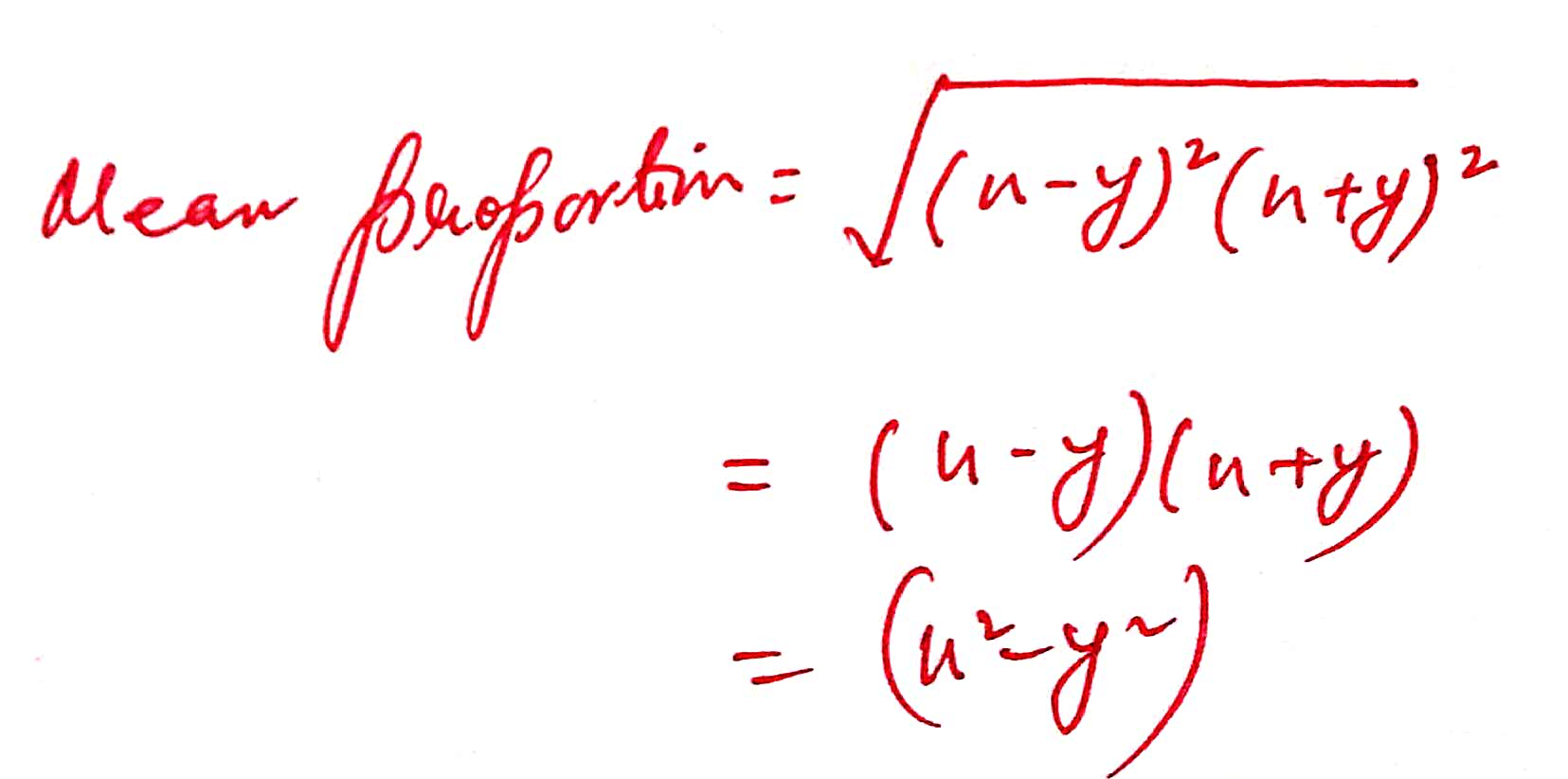33 ) Find the mean proportion of the numbers 1/12 and 1/75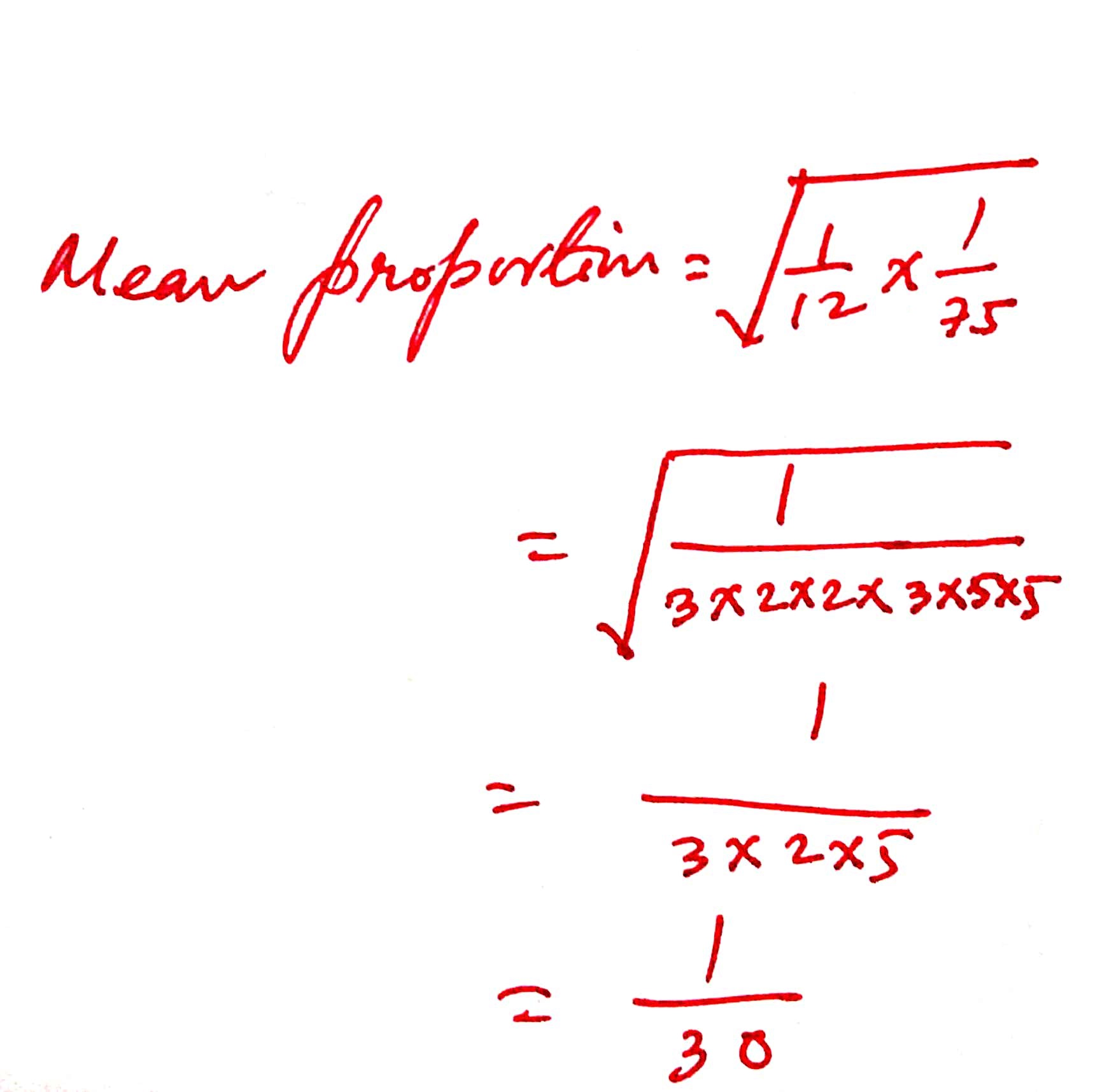34 ) Find the mean proportion of the numbers 0.5 and 4.5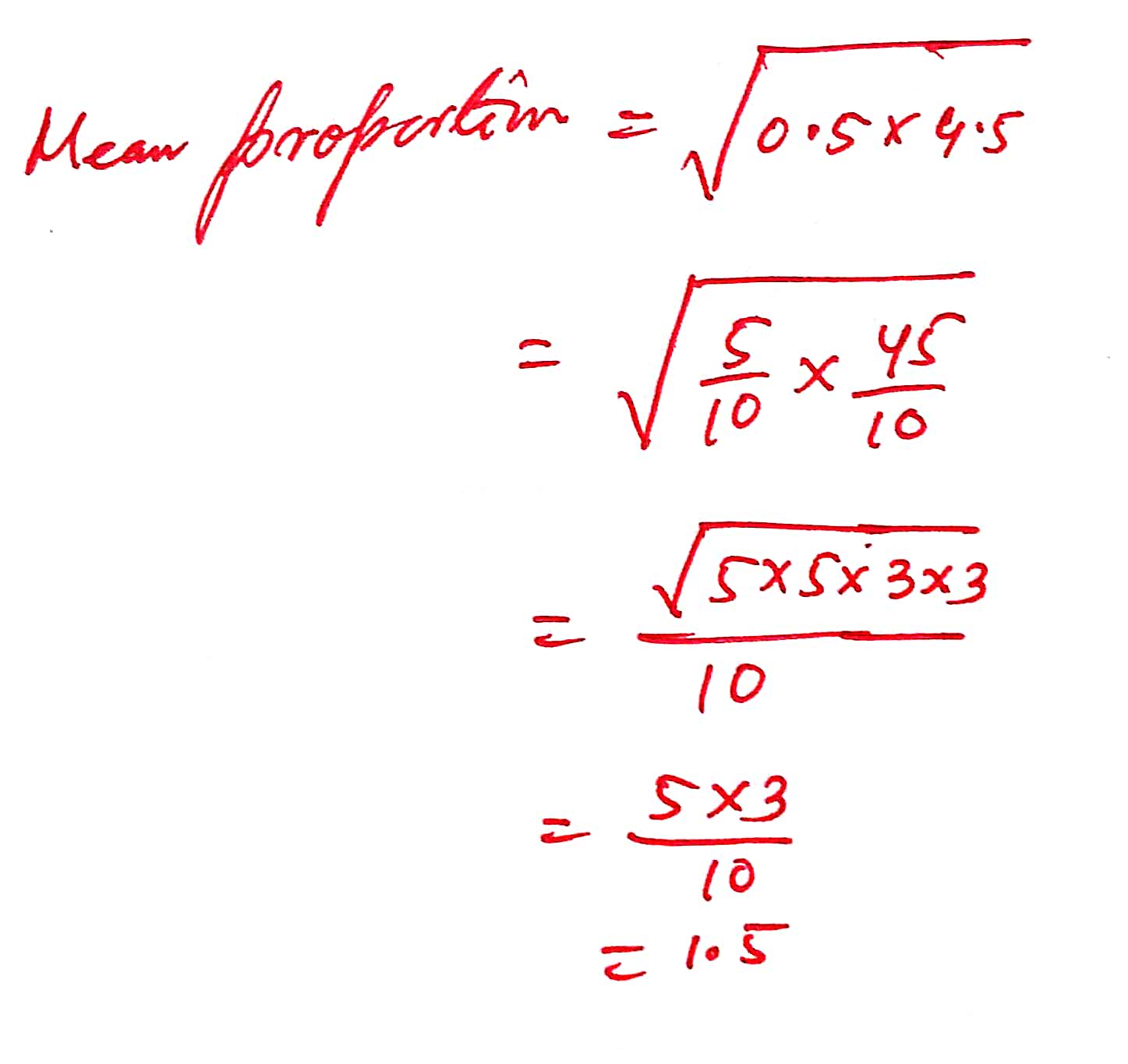35) Find the mean proportion of the numbers pqr and pr/q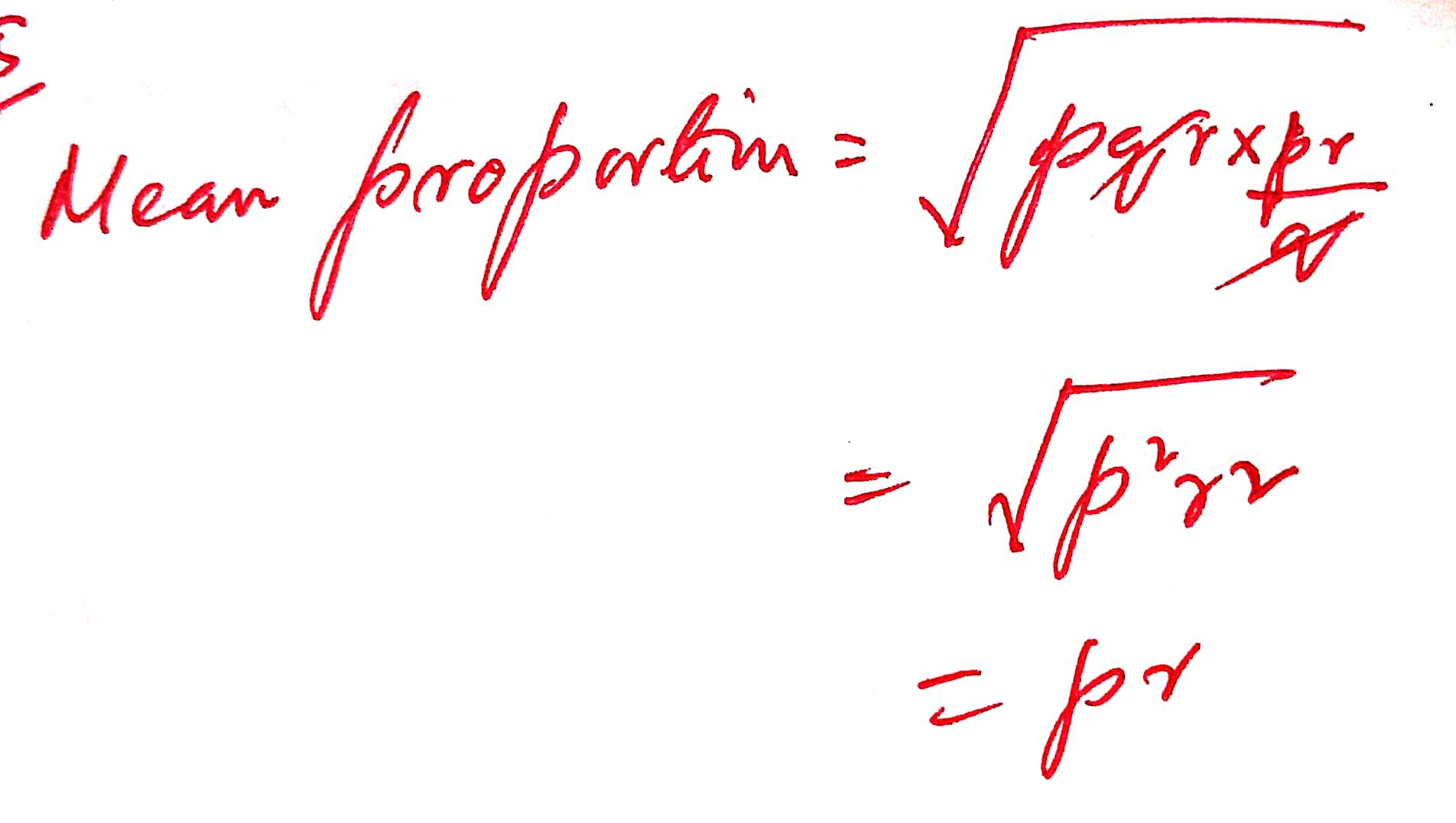36 ) Find the mean proportion of the numbers xy2 and xz2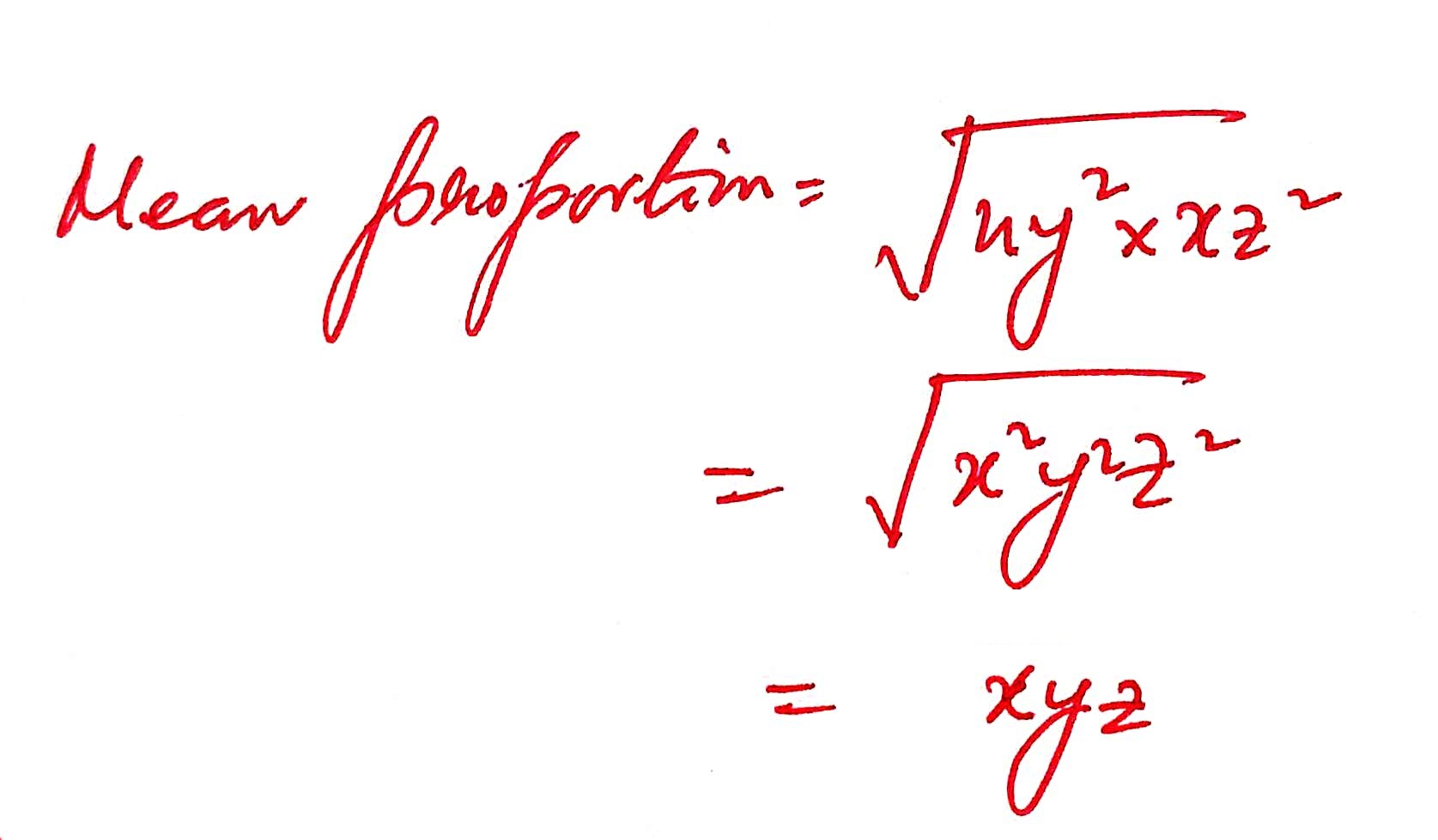37 ) Find the mean proportion of the numbers ( 6 +3 3) and ( 8 – 43 )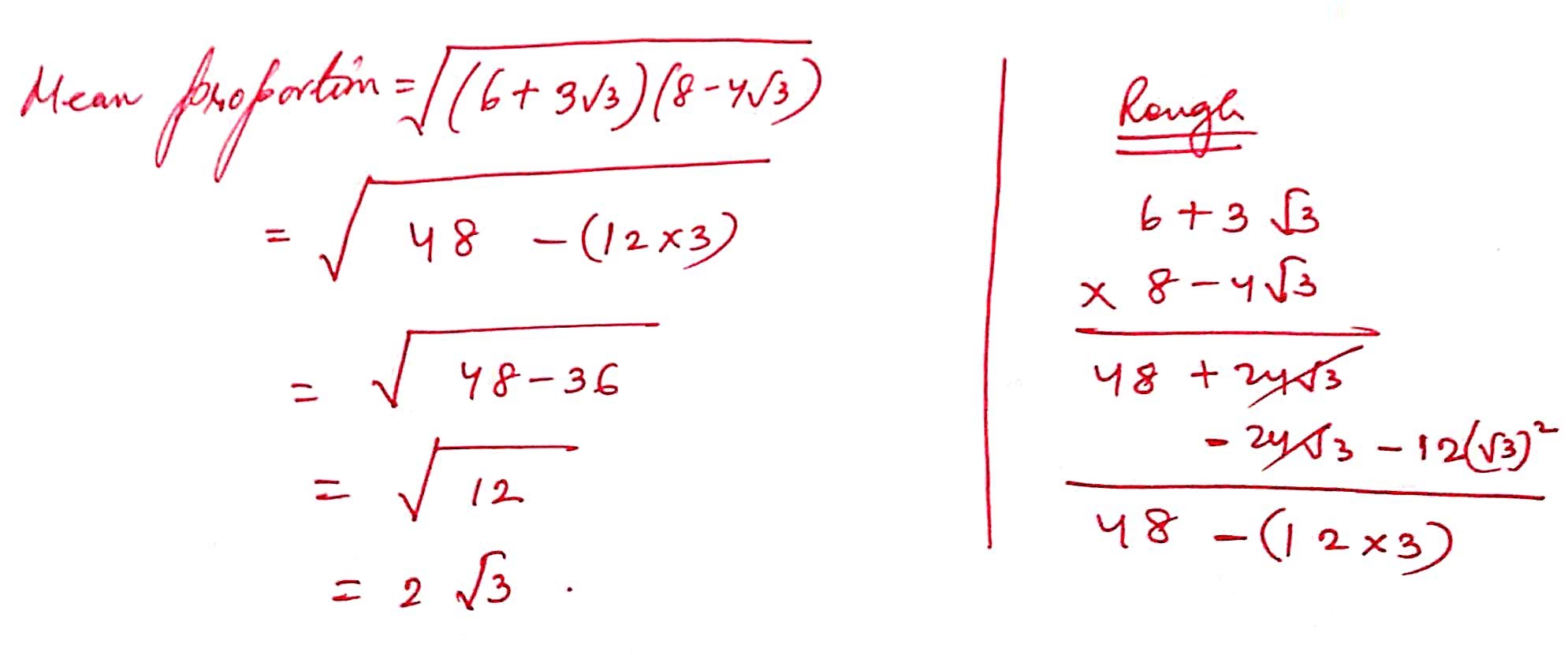38 ) Find the mean proportion of the numbers (a – b) and (a3 – a2b)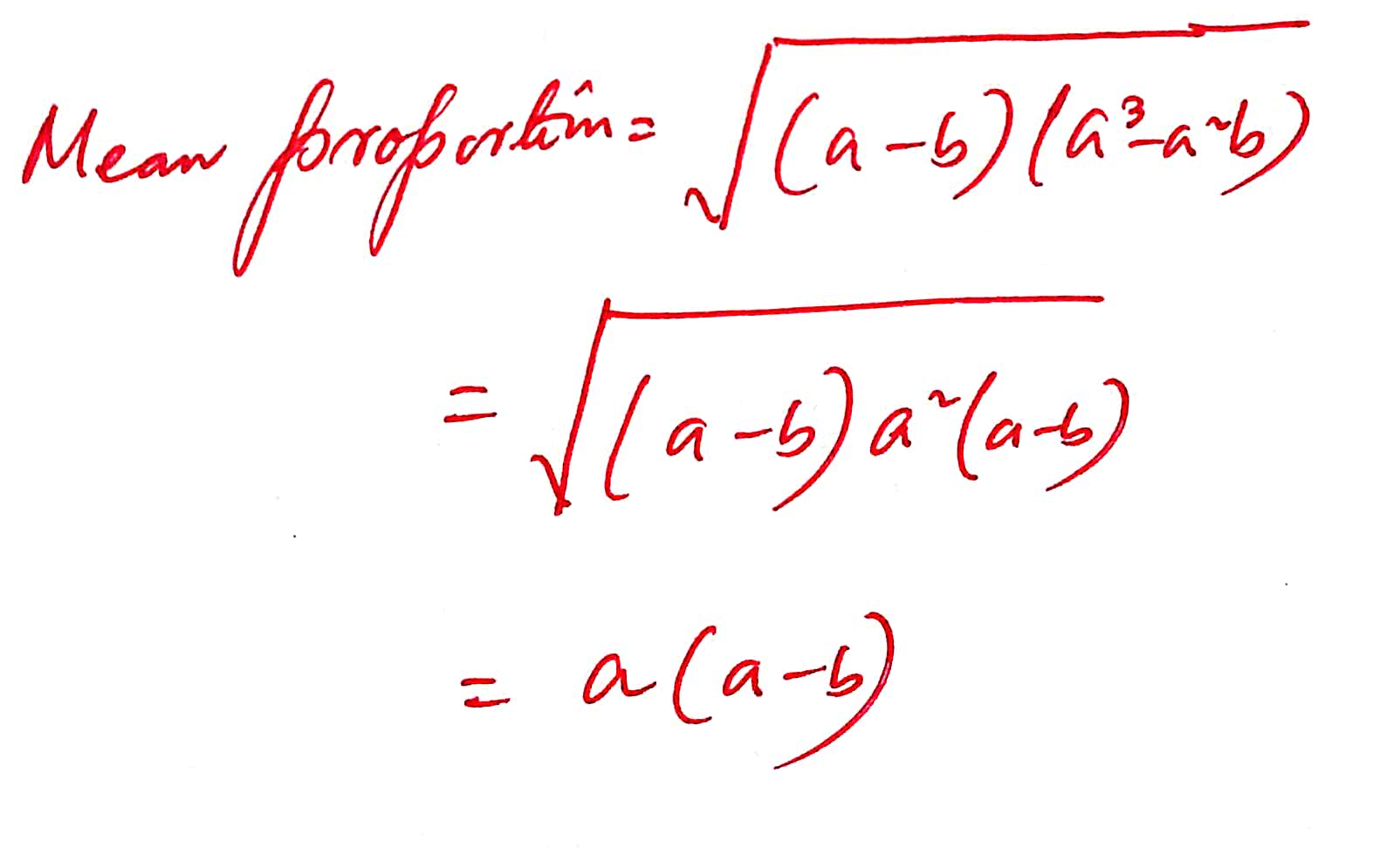39) 6 is the mean proportion between two numbers x and y and 48 is third propotion to x and y. Find the numbers.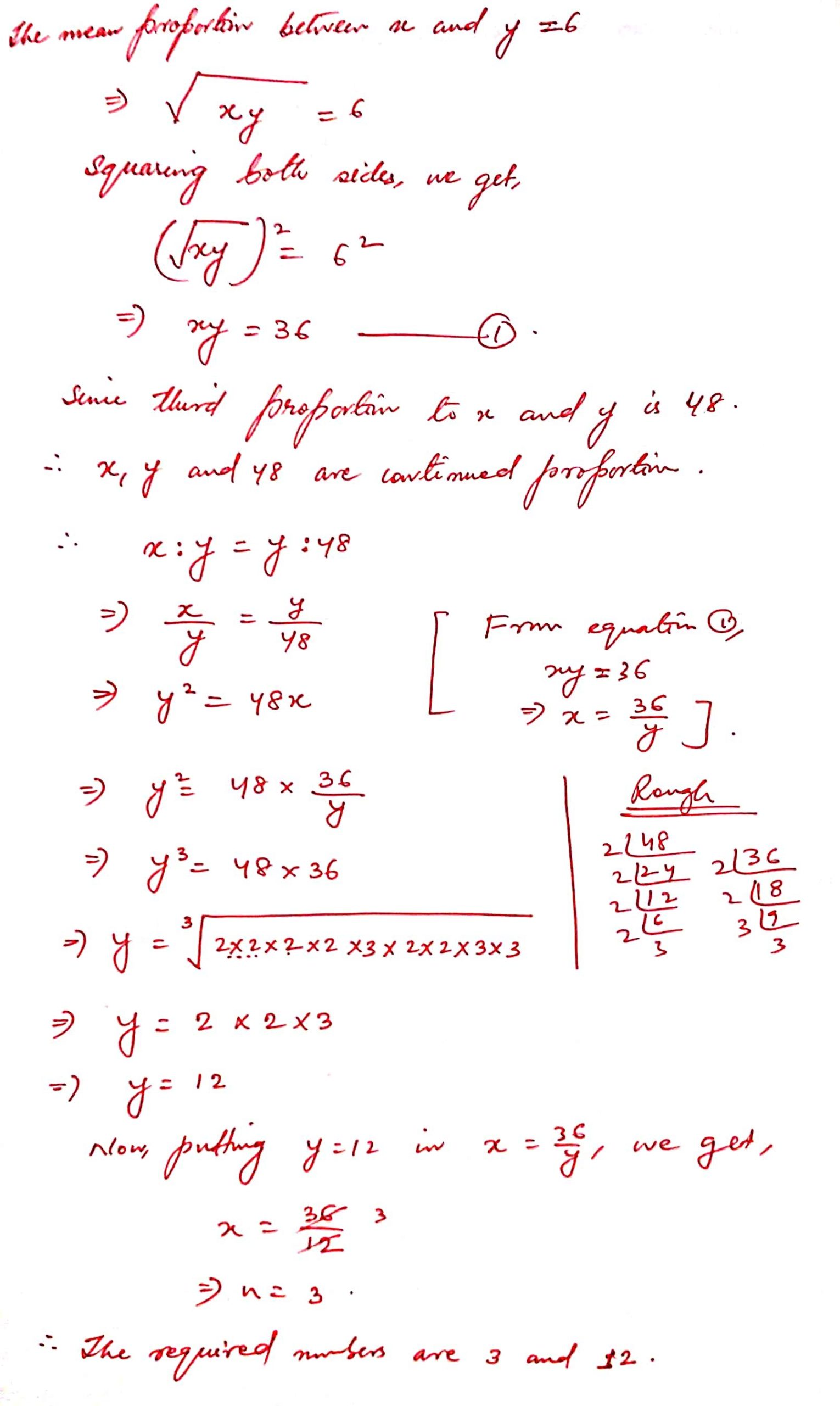40 ) What least number must be added to each of the numbers 6,15,20 and 43 to make them proportional ?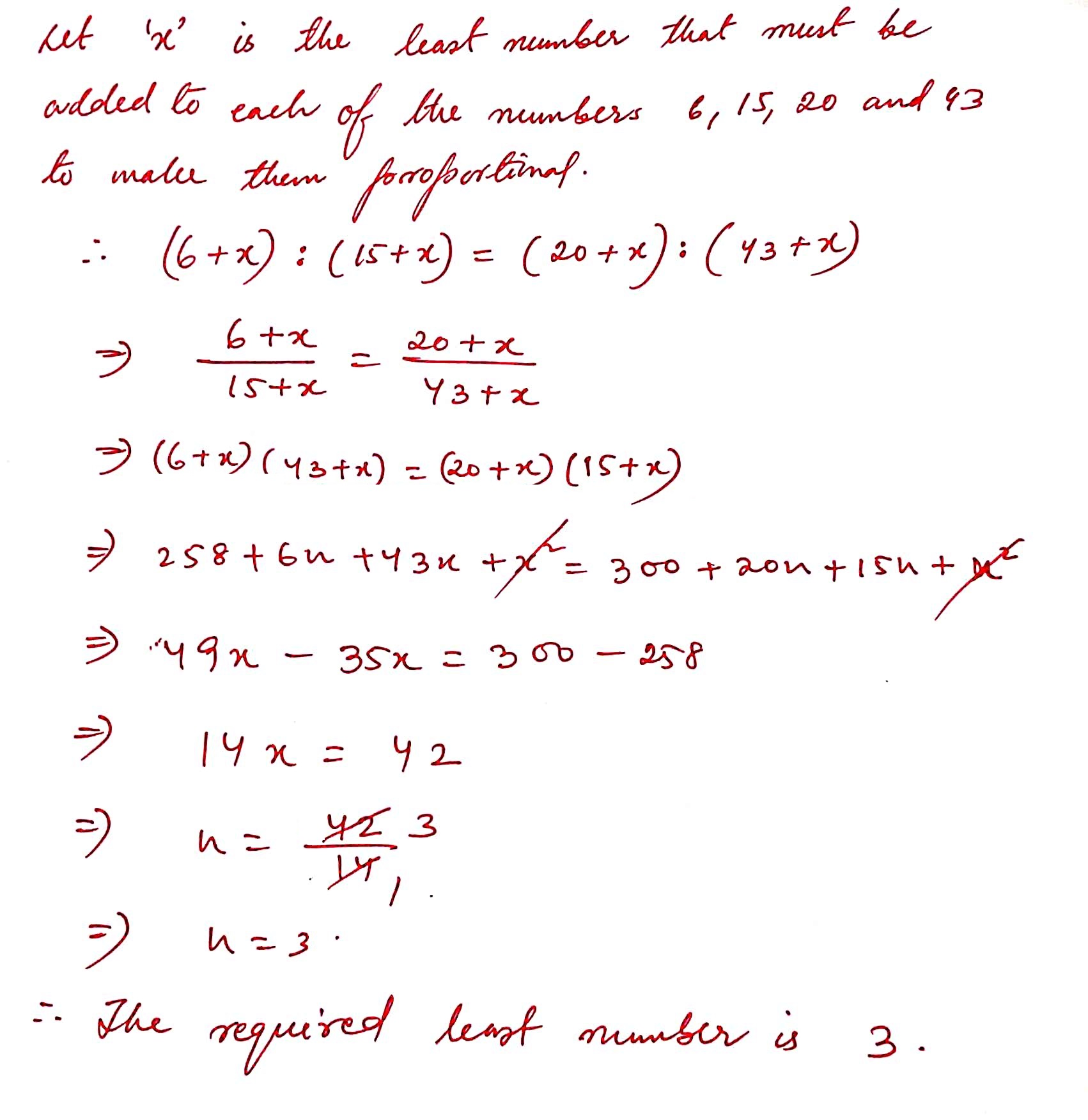41 ) If ( x + 5 ) is the mean proportion between ( x + 2 ) and ( x + 9 ), find the value of x.